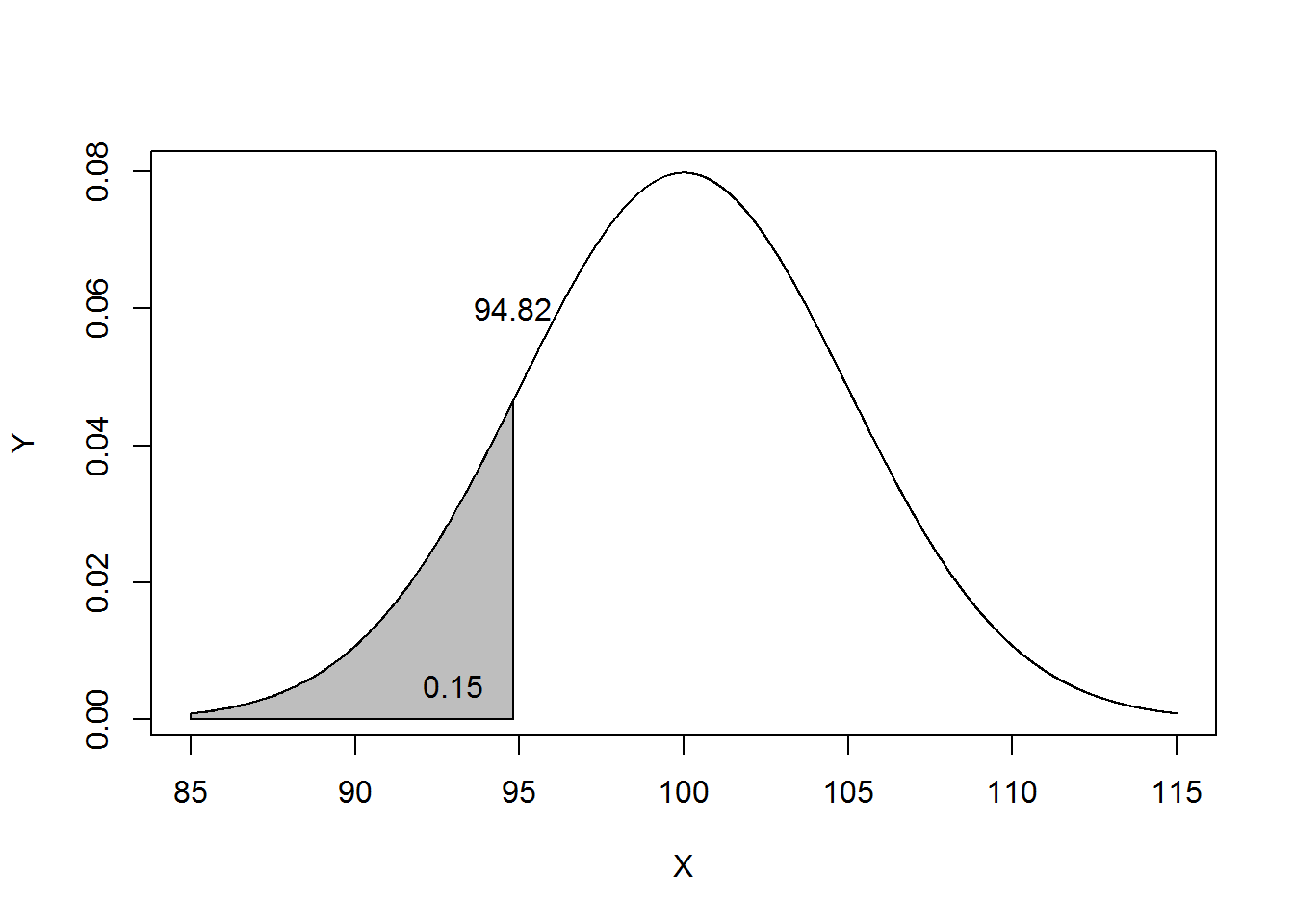load("../data/datasets_L08.Rda")

# It is often desirable to generate fake data. Sometimes we just want data to
# play around with. Other times we want to generate data similar to what we
# expect to collect to see if our proposed analysis works as expected. Or we
# want to simulate data collection many times over in order to estimate a
# statistical measure such as standard error.

# sampling data -----------------------------------------------------------

# An easy way to generate data is to sample from existing data. The sample
# function makes this possible. The syntax is sample(x, size, replace) where x
# is either a vector of one or more elements from which to choose, or a positive
# integer, size is a non-negative integer giving the number of items to choose,
# and replace is a logical setting about whether sampling should be with
# replacement (The default is FALSE).

# The most basic use is to generate a random permutation of the numbers 1:n:
sample(5) # sample without replacement
##  5 2 3 4 1
# or generate a random permutation of a vector:
dat <- c(10,12,18,16,18,9)
sample(dat)
##  18  9 10 18 16 12
# bootstrap resampling: sampling the same number of items WITH replacement
sample(dat, replace = TRUE) 
##   9 18 18 12  9 18
# Using set.seed() allows us to reproduce the same random sample. Just give it a
# whole number, any number.
set.seed(2)
sample(10)
##    2  7  5 10  6  8  1  3  4  9
set.seed(2)
sample(10)
##    2  7  5 10  6  8  1  3  4  9
# The size argument allows to select a certain number of elements from a vector.
# For example, sample 10 states:
sample(state.abb, size = 10)
##   "NV" "ID" "OR" "FL" "ME" "RI" "TX" "GA" "VA" "AR"
# Using 1:6 and size=1, we can simulate the roll of a die:
sample(1:6, size=1)
##  4
# We can simulate the roll of a die 100 times by setting size=100 and
# replace=TRUE
sample(1:6, size=100, replace=TRUE)
##    3 6 1 3 3 1 3 6 1 1 1 5 6 4 4 6 2 5 1 6 2 1 1 6 5 6 3 4 5 1 1 5 6 2 5
##   5 6 4 5 5 6 4 2 6 3 3 3 2 1 2 2 1 2 2 5 2 6 3 4 3 5 1 3 2 6 6 2 5 3 6
##   3 3 4 3 2 3 1 1 3 2 3 6 5 2 4 6 3 1 1 1 5 3 4 5 5 6 1 6 4 1
# sample produces a vector, so we can manipulate it as we would any other
# vector. For example, simulate a 100 die rolls and tally up the totals using
# table() and prop.table():
prop.table(table(sample(1:6, size=100, replace=TRUE)))
##
##    1    2    3    4    5    6
## 0.17 0.26 0.12 0.13 0.13 0.19
# using the forward-pipe operator: %>%
library(magrittr)
sample(1:6, size=100, replace=TRUE) %>% table() %>% prop.table()
## .
##    1    2    3    4    5    6
## 0.16 0.16 0.16 0.13 0.21 0.18
# Or simulate rolling two dice and summing the total:
sum(sample(1:6, size=2, replace=TRUE))
##  3
# same thing with %>%
sample(6, size=2, replace=TRUE) %>% sum()
##  4
# We can use the replicate() function to replicate samples. The replicate()
# function allows you to replicate an expression as many times as you specify.
# The basix syntax is replicate(n, expr) where n is the number of replications
# and expr is the expression you want to replicate.

# Roll 2 dice and keep the largest number, 10,000 times:
rolls <- replicate(n=1e5, expr = max(sample(1:6, size=2, replace=TRUE)))
# calculate proportions:
prop.table(table(rolls))
## rolls
##       1       2       3       4       5       6
## 0.02763 0.08287 0.13703 0.19466 0.25044 0.30737
barplot(table(rolls))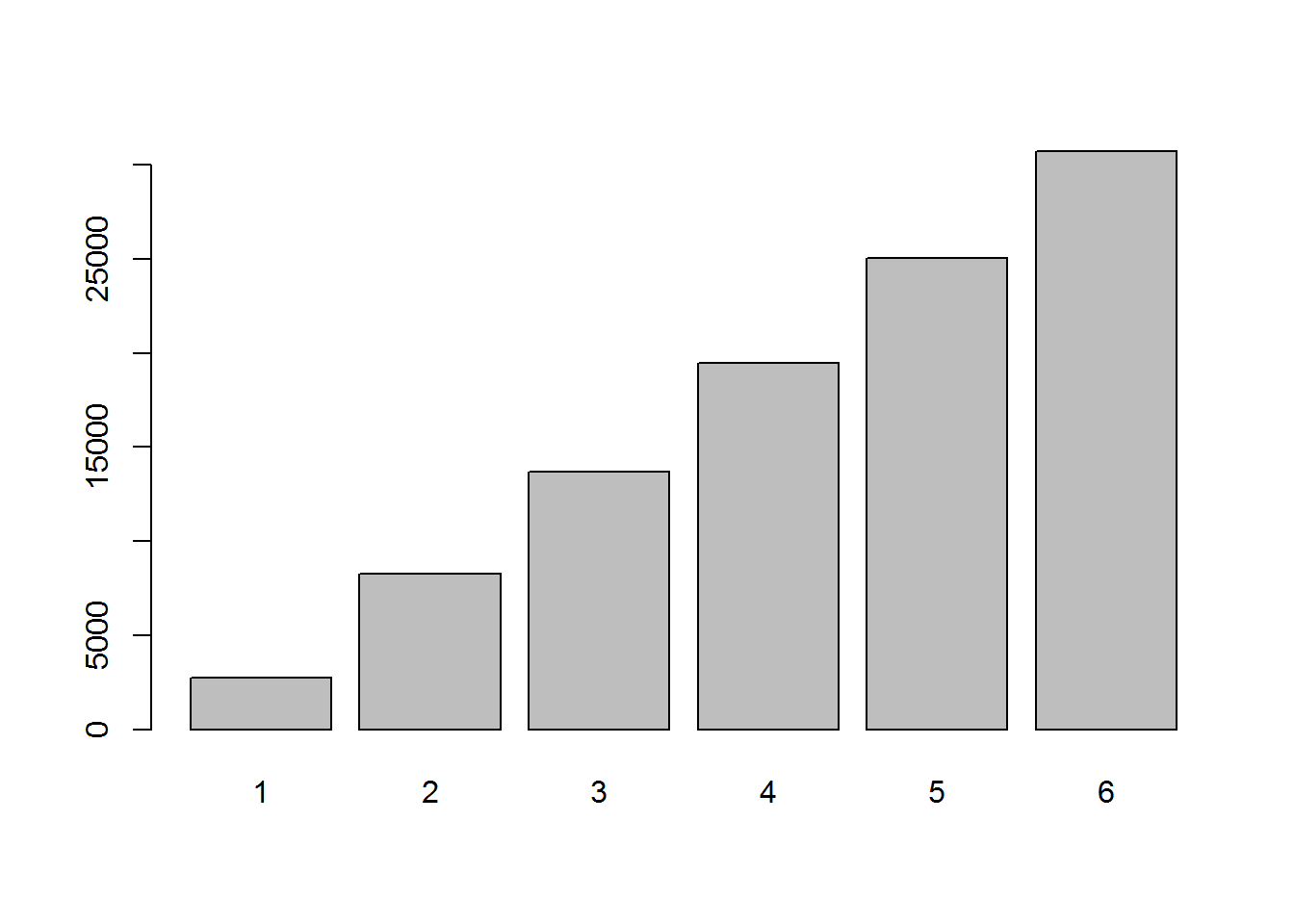rm(rolls)

# The sample function also has a prob argument that allows you to assign
# probabilities to your items. For example to simulate the flip of a loaded
# coin, with Tails having probability 0.65:
flips <- sample(c("H","T"), 1000, replace=TRUE, prob = c(0.35,0.65))
prop.table(table(flips))
## flips
##     H     T
## 0.344 0.656
rm(flips)

# Coins are nice, but we can also use sample to generate practical data, for
# example males and females. The following web site says UVa has 11,632 female
# students and 10,353 male students, as of Fall 2015:

# https://avillage.web.virginia.edu/iaas/instreports/studat/hist/enroll/school_by_gender.shtm
uva <- c(11632, 10353) # female, male
round(uva/sum(uva),2)
##  0.53 0.47
# We can generate a fake random sample of 500 UVa students with a weighted sampling
# scheme like so:
students <- sample(c("female","male"), 500, replace=TRUE, prob = c(0.53, 0.47))
prop.table(table(students))
## students
## female   male
##  0.548  0.452
rm(students, uva)

# When used with subsetting brackets, sample() can be used to create training
# and test sets. For example, say we want to build some sort of predictive model
# using our training data. We may want to use half our data to build the model
# and then use the other half to evaluate its performance.
train <- sample(nrow(weather), size= nrow(weather)/2)

# train is a random sample of numbers from 1 - 365. We can treat these like row
# numbers.

weatherTrain <- weather[train,]
weatherTest <- weather[-train,]
# confirm no intersection
dplyr::intersect(weatherTrain, weatherTest) 
##   Month                     EST
##   Max.TemperatureF          Mean.TemperatureF
##   Min.TemperatureF          freezing
##   Max.Dew.PointF            MeanDew.PointF
##   Min.DewpointF             Max.Humidity
##  Mean.Humidity             Min.Humidity
##  Max.Sea.Level.PressureIn  Mean.Sea.Level.PressureIn
##  Min.Sea.Level.PressureIn  Max.VisibilityMiles
##  Mean.VisibilityMiles      Min.VisibilityMiles
##  Max.Wind.SpeedMPH         Mean.Wind.SpeedMPH
##  Max.Gust.SpeedMPH         PrecipitationIn
##  Cloud.Cover.Index         Events
##  Temp.Range                humidity.range
##  Mean.TemperatureCZ        Mean.TemperatureC
##  Cold.Rank                 snow
##  Date                      Total.Precip.Month
## <0 rows> (or 0-length row.names)
# generating fixed levels -------------------------------------------------

# Often generating data means creating a series of fixed levels, such as 10
# males and 10 females. The rep() function can be useful for this. Below we
# replicate 10 each of "M" and "F":
rep(c("M","F"), each=10)
##   "M" "M" "M" "M" "M" "M" "M" "M" "M" "M" "F" "F" "F" "F" "F" "F" "F"
##  "F" "F" "F"
# we can also specify number of times the vector is replicated:
rep(c("M","F"), times=10)
##   "M" "F" "M" "F" "M" "F" "M" "F" "M" "F" "M" "F" "M" "F" "M" "F" "M"
##  "F" "M" "F"
# Finally we can replicate until a certain length is achieved
rep(c("M","F"), length.out = 15)
##   "M" "F" "M" "F" "M" "F" "M" "F" "M" "F" "M" "F" "M" "F" "M"
# or just length, for short
rep(c("M","F"), length = 15)
##   "M" "F" "M" "F" "M" "F" "M" "F" "M" "F" "M" "F" "M" "F" "M"
# Notice that all these generated a character vector. To use as a "factor", we
# would need to wrap it in the factor() function.
factor(rep(c("M","F"), each=10))
##   M M M M M M M M M M F F F F F F F F F F
## Levels: F M
# A function specifically for creating factors is the gl() function. gl =
# "generate levels". Below we generate a factor with 2 levels of 10 each and
# labels of "M" and "F". Notice the result is a factor.
gl(n = 2, k = 10, labels = c("M","F"))
##   M M M M M M M M M M F F F F F F F F F F
## Levels: M F
# A more common occurence is combinations of fixed levels, say gender,
# education, and status. A function that helps create every combination of
# levels is expand.grid(). Below we generate every combination of the levels
# provided for gender, education, and status. Notice the first factors vary
# fastest.
expand.grid(gender=c("M","F"),
education=c("HS","College","Advanced"),
status=c("Single","Married","Divorced","Widowed"))
##    gender education   status
## 1       M        HS   Single
## 2       F        HS   Single
## 3       M   College   Single
## 4       F   College   Single
## 5       M  Advanced   Single
## 6       F  Advanced   Single
## 7       M        HS  Married
## 8       F        HS  Married
## 9       M   College  Married
## 10      F   College  Married
## 11      M  Advanced  Married
## 12      F  Advanced  Married
## 13      M        HS Divorced
## 14      F        HS Divorced
## 15      M   College Divorced
## 16      F   College Divorced
## 17      M  Advanced Divorced
## 18      F  Advanced Divorced
## 19      M        HS  Widowed
## 20      F        HS  Widowed
## 21      M   College  Widowed
## 22      F   College  Widowed
## 23      M  Advanced  Widowed
## 24      F  Advanced  Widowed
# Notice that creates a data frame that we can save:
DF <- expand.grid(gender=c("M","F"),
education=c("HS","College","Advanced"),
status=c("Single","Married","Divorced","Widowed"))
class(DF)
##  "data.frame"
rm(DF)

# Extended example --------------------------------------------------------

# Create a experimental design plan and write out to a csv file.

# In this experiment, 3 people throw 3 different kinds of paper airplanes, made of 3
# paper types (3x3 = 9 planes), throwing each plane 8 times.

# > 3*3*3*8
#  216

schedule <- expand.grid(thrower=c("Clay","Rod","Kevin"),
paper=c("18", "20", "24"),
design=c("a","b","c"),
rep=1:8)

# Randomize and drop the rep column. The sample(nrow(schedule)) code scrambles
# the numbers 1 through 216, which I then use to randomly shuffle the schedule
# of throws.
k <- sample(nrow(schedule))
schedule <- schedule[k,1:3]
head(schedule, n = 10)
##     thrower paper design
## 174   Kevin    18      b
## 82     Clay    18      a
## 96    Kevin    20      b
## 211    Clay    20      c
## 89      Rod    24      a
## 26      Rod    24      c
## 158     Rod    20      c
## 136    Clay    18      a
## 58     Clay    20      a
## 91     Clay    18      b
# output to csv file for logging "distance flown" data
write.csv(schedule, file="throwLog.csv", row.names=FALSE)

# generating numerical sequences ------------------------------------------

# The seq() function allows you to generate sequences of numbers:
seq(from = 0, to = 10, by = 2)
##   0  2  4  6  8 10
seq(0, 10, 0.2)
##    0.0  0.2  0.4  0.6  0.8  1.0  1.2  1.4  1.6  1.8  2.0  2.2  2.4  2.6
##   2.8  3.0  3.2  3.4  3.6  3.8  4.0  4.2  4.4  4.6  4.8  5.0  5.2  5.4
##   5.6  5.8  6.0  6.2  6.4  6.6  6.8  7.0  7.2  7.4  7.6  7.8  8.0  8.2
##   8.4  8.6  8.8  9.0  9.2  9.4  9.6  9.8 10.0
# go backwards:
seq(1000, 0, -100)
##   1000  900  800  700  600  500  400  300  200  100    0
# The seq() function has a length.out argument that allows you to specify the
# size of the vector you want to create. It automatically calculates the
# increment. We usually just abbreviate to length
seq(1, 10, length = 30)
##    1.000000  1.310345  1.620690  1.931034  2.241379  2.551724  2.862069
##    3.172414  3.482759  3.793103  4.103448  4.413793  4.724138  5.034483
##   5.344828  5.655172  5.965517  6.275862  6.586207  6.896552  7.206897
##   7.517241  7.827586  8.137931  8.448276  8.758621  9.068966  9.379310
##   9.689655 10.000000
# The colon operator(:) also allows you to generate regular sequences in steps
# of 1.
1:10
##    1  2  3  4  5  6  7  8  9 10
10:-10 # reverse direction
##    10   9   8   7   6   5   4   3   2   1   0  -1  -2  -3  -4  -5  -6
##   -7  -8  -9 -10
# When used with factors, the colon operator generates an interaction factor:
f1 <- gl(n = 2, k = 3); f1
##  1 1 1 2 2 2
## Levels: 1 2
f2 <- gl(n = 3, k = 2, labels = c("a","b","c")); f2
##  a a b b c c
## Levels: a b c
f1:f2 # a factor, the "cross"  f1 x f2
##  1:a 1:a 1:b 2:b 2:c 2:c
## Levels: 1:a 1:b 1:c 2:a 2:b 2:c
rm(f1, f2)

# Two related functions are seq_along() and seq_len(). seq_along() returns the
# indices of a vector while seq_len(n) returns an integer vector of 1:n.
seq_along(100:120)
##    1  2  3  4  5  6  7  8  9 10 11 12 13 14 15 16 17 18 19 20 21
seq_along(state.abb) # state.abb = built-in vector of state abbreviations
##    1  2  3  4  5  6  7  8  9 10 11 12 13 14 15 16 17 18 19 20 21 22 23
##  24 25 26 27 28 29 30 31 32 33 34 35 36 37 38 39 40 41 42 43 44 45 46
##  47 48 49 50
seq_len(10)
##    1  2  3  4  5  6  7  8  9 10
# generating random data from a probability distribution ------------------

# A central idea in inferential statistics is that the distribution of data can
# often be approximated by a theoretical distribution. R provides functions for
# working with several well-known theoretical distributions, including the
# ability to generate data from those distributions. One we've used several
# times in the lectures is the rnorm() function which generates data from a
# Normal distribution.

# In R, the functions for theoretical distributions take the form of dxxx, pxxx,
# qxxx and rxxx.

# - dxxx is for the probability density/mass function (dnorm)
# - pxxx is for the cumulative distribution function (pnorm)
# - qxxx is for the quantile function (qnorm)
# - rxxx is for random variate generation (rnorm)

# For this lecture we're interested in the rxxx variety. See the lecture
# appendix for a review of the others. See help(Distributions) for all
# distributions available with base R.

# Draw random values from a theoretical distribution.
# 10 random draws from N(100,5)
rnorm(n = 10, mean = 100, sd = 5)
##   100.51617 103.09315 102.02745 102.80894  98.70051 101.65664 107.06122
##   106.18988 100.97244 101.95004
# 10 random draws from b(1,0.5)
# AKA, 10 coin flips
rbinom(n = 10, size = 1, prob = 0.5)
##   0 1 0 0 0 0 0 0 0 1
# 10 random draws from b(1,0.8)
# AKA, 10 coin flips with a coin loaded Heads (or Tails) 80% of time
rbinom(n = 10, size = 1, prob = 0.8)
##   1 1 1 1 1 1 1 1 1 0
# 10 random draws from b(10,0.5)
# AKA, 10 results of 10 coin flips
rbinom(n = 10, size = 10, prob = 0.5)
##   4 7 6 6 5 4 5 3 8 3
# We can use a binomial distribution to simulate dichotmous answers such as
# Yes/No or success/fail. Simulate a vector of responses where respondents are
# 65% likely to say Yes (1) versus No (0)
rbinom(n = 10, size = 1, prob = 0.65)
##   0 1 0 1 0 1 0 0 0 1
# could also just use sample
sample(c("Y","N"), size = 10, replace = TRUE, prob = c(.65, .35))
##   "N" "Y" "Y" "N" "Y" "Y" "Y" "Y" "Y" "Y"
# 10 random draws from a uniform distribution u(0,100)
runif(10,0,100)
##   20.19432 22.49488 57.68923 96.62367 47.88132 28.21963 84.44360
##   16.09101 70.68120 42.15722
# A uniform distribution can be good for random sampling. Let's say we want to
# sample about 10% of our SenateBills data:
k <- runif(nrow(SenateBills),0,1) # [0,1] interval is default
sbSamp <- SenateBills[k < 0.1, ] # sample about 10% of rows
dim(sbSamp)
##  294   4
# dplyr does this as well without the need for runif; and it's precise in its
# sampling fraction.
sbSamp <- dplyr::sample_frac(SenateBills, 0.1) # sample exactly 10% of rows
dim(sbSamp)
##  302   4
rm(sbSamp, k)

# The arguments to rxxx functions can take vectors! This means we can use one
# function call to generate draws from multiple distributions.

# alternating random values from N(10,4) and N(100,40)
rnorm(10, mean = c(10,100),sd = c(4,40))
##    12.458031  73.389950  16.151974  11.171337  15.014355 156.455648
##    14.467368 105.869758   5.178173 185.017624
# 30 random draws, 10 each from N(10,4), N(90,4) and N(400,4)
rnorm(30, mean = rep(c(10,90,400),each=10), sd = 4)
##     9.727233  13.365185   7.314122   7.883727  12.540061  15.782295
##    12.947664  10.925502  11.997381  12.056117  95.230665  89.098066
##   96.628958  98.927913  87.428768  88.617991  85.466042  97.464190
##   90.744968  94.643161 397.752502 393.384465 395.693224 396.064585
##  398.361146 400.400153 397.171173 398.315040 394.907753 394.961825
# 100 random draws, 50 each from b(5,0.5) and b(50,0.5)
rbinom(n = 100, size = rep(c(5,50),each=50), prob = 0.5)
##     2  2  1  3  2  3  2  2  2  2  3  3  3  3  2  3  3  3  3  3  4  2  1
##    3  1  5  1  3  1  3  1  2  0  2  3  2  4  2  0  0  2  3  2  1  1  5
##    2  3  1  1 29 24 20 22 22 25 29 22 23 22 24 26 23 22 26 29 22 30 23
##   32 20 27 25 22 27 21 28 28 26 28 19 27 24 30 22 25 26 20 29 28 26 25
##   21 25 29 17 29 26 27 25
# Combined with matrix(), one can generate "multiple" random samples from a
# distribution. For example, draw 5 random samples of size 10 from a N(10,1):
matrix(rnorm(10*5,10,1),ncol=5)
##            [,1]      [,2]      [,3]      [,4]      [,5]
##  [1,] 11.818092 11.501619  9.650670  8.563924  9.881488
##  [2,]  9.402527 11.275014  9.725050  9.079653 10.482335
##  [3,] 11.609256 10.365992  9.763614 10.500186 11.330261
##  [4,] 10.740648  8.978442 10.607858 10.050714 10.521051
##  [5,]  7.835578 11.013852  8.485332 10.299846 10.685468
##  [6,] 10.729999 10.865401 10.205393 10.522541  8.999784
##  [7,]  9.405597 10.076220  9.380070  9.576029 11.041871
##  [8,]  9.934175 10.215511 10.851907 11.321348 10.666949
##  [9,] 10.025773 10.860156  9.762195 12.033644  8.106476
## [10,]  9.244122 10.228131 10.475144 10.973959 10.045625
# Technically we drew one sample of size 50 and then laid it out in a 10x5
# matrix.

# Using ifelse() we can generate different data based on a TRUE/FALSE condition.
# Let's say we have treated and untreated subjects. I'd like to generate Normal
# data that differs based on the treatment.
trtmt <- sample(c("Treated","Untreated"), size = 20, replace = TRUE)
ifelse(trtmt=="Treated", yes = rnorm(20, 10, 1), no = rnorm(20, 20, 1))
##   10.173661 10.073728  9.763664  9.989032 10.421568 10.059920 10.127133
##    9.505456 19.214323  8.119144 20.382744 19.225438 18.356364  9.323236
##  20.978069  8.824862 10.376644  9.396099 20.939601 10.143976
# Notice we have to make the length of the yes/no arguments the SAME LENGTH as
# the trtmt=="Treated" logical vector! What happens if we use rnorm(n=1,...)?

# What about more than two groups?
n <- 200
trtmt <- sample(LETTERS[1:6], size = n, replace = TRUE)

# Say we want to generate differnt Normal data for each group. One way is to do
# a for-loop with multiple if statements:

val <- numeric(n) # empty vector
for(i in seq_along(trtmt)){
if(trtmt[i]=="A") val[i] <- rnorm(1, 10, 2)
else if(trtmt[i]=="B") val[i] <- rnorm(1, 20, 4)
else if(trtmt[i]=="C") val[i] <- rnorm(1, 30, 6)
else if(trtmt[i]=="D") val[i] <- rnorm(1, 40, 8)
else if(trtmt[i]=="E") val[i] <- rnorm(1, 50, 10)
else val[i] <- rnorm(1, 60, 12)
}
val
##    25.178157 67.783169 54.699414 17.340495 21.368806 27.277365 47.786011
##    23.309206 63.743062 12.019384 34.054124 30.321399 14.257338 46.447698
##    7.153634 41.809258 24.596105 27.567158 47.902190 47.721632 94.813795
##   34.183122 15.597612 49.394595 28.407936 13.923586 18.692210 30.439670
##   42.093173 60.956933 20.881782 20.725780 33.284687 38.009420 21.969867
##   18.178201 37.461436 11.259505 79.219147 25.772086 44.860551 48.511650
##   10.226086 44.604392 10.928839 90.869261 40.103735 56.145175 39.651682
##   73.148289 49.204968 10.999851  9.589315 10.440449 25.392600 17.070274
##   53.987465 45.061611 42.069700 57.141754 58.090823  7.183850 48.334189
##   53.451762 67.959244 80.455027 13.500113 23.692508 24.057468  7.706210
##   26.430870 39.939059 74.529946 43.848131 43.722571 58.362669 42.755058
##    8.829214 25.455885 57.304359  9.923464 36.753192 55.857044 47.270945
##   38.457511  8.515536 17.883682 42.067665 56.865547 41.789596 44.423289
##   55.154916 56.344616 55.763583 27.406531 13.215162 64.536255 46.371342
##   62.457110 46.588639 51.919005 44.700642 17.906851 44.865921  7.175344
##  62.907137 63.071289 18.641895 73.337352 65.954085 57.548947 20.306832
##  50.383075 22.667854  8.572592 54.953157 22.550881 49.350136 38.469135
##  19.440509 36.397031 10.578477  7.863461 27.726026 49.181323 35.323055
##  38.932857  8.972407 47.711257 14.403581 50.752293 34.910021 24.911141
##  37.175757 62.122042 37.013150 93.315776 54.395980 12.678741 41.474396
##  71.646747 20.161215 56.056748 38.124600 10.031464 10.897841 22.020368
##  13.285414 60.150495 11.087281 56.167425 11.481099 37.617251  8.046307
##  23.383099  8.617180 34.882177  9.038220 60.421583 48.416087 44.130659
##  22.462608 27.950448 65.663063 51.672350 11.966499 45.733809 74.767146
##  31.937591 33.566627 52.222168 21.470502 23.805968 55.670395 60.120510
##  29.440410 20.224699 47.346326 12.163583 57.899855 55.719336 28.997240
##  24.320988  9.796929 65.911099 29.899361 61.760759 14.097528 21.504321
##  41.364945 34.903663 58.217159 83.225570 50.829490 65.163061 50.011882
##  29.712996 49.366124 42.797344 10.909823
# A more R-like way would be to take advantage of vectorized functions. First
# create a data frame with one row for each group and the mean and standard
# deviations we want to use to generate the data for that group.
dat <- data.frame(g=LETTERS[1:6],mean=seq(10,60,10),sd=seq(2,12,2))

# Now sample the row numbers (1 - 6) WITH replacement. We can use these to
# randomly sample the data frame rows. Recall that we can repeatedly call a row
# or element using subsetting brackets. For example, call the first row of
# allStocks 10 times:
allStocks[c(1,1,1,1,1),]
##           Date  Open  High   Low Close  Volume Stock Day Month Change
## 1   2014-03-26 67.76 68.05 67.18 67.25 1785164  bbby Wed   Mar  -0.51
## 1.1 2014-03-26 67.76 68.05 67.18 67.25 1785164  bbby Wed   Mar  -0.51
## 1.2 2014-03-26 67.76 68.05 67.18 67.25 1785164  bbby Wed   Mar  -0.51
## 1.3 2014-03-26 67.76 68.05 67.18 67.25 1785164  bbby Wed   Mar  -0.51
## 1.4 2014-03-26 67.76 68.05 67.18 67.25 1785164  bbby Wed   Mar  -0.51
# Let's exploit that to randomly sample with replacement our data frame of
# groups:
k <- sample(1:6, n, replace = TRUE)
dat <- dat[k,]

# Now generate our data for each group using ONE call to rnorm.
dat$vals <- rnorm(n, mean=dat$mean, sd=dat$sd) head(dat) ## g mean sd vals ## 1 A 10 2 7.615936 ## 2 B 20 4 21.648727 ## 3 C 30 6 27.289520 ## 1.1 A 10 2 10.314583 ## 6 F 60 12 56.483305 ## 1.2 A 10 2 11.270186 # A demonstration of the Central Limit Theorem ---------------------------- # The Central Limit Theorem states that the sum of a large number of independent # random variables will be approximately normally distributed almost regardless # of their individual distributions. We can demonstrate this using various rxxx # functions. # sum 6 values from 6 different distributions (sample size = 6) n <- 1e4 # simulate 1000 times clt <- rexp(n, rate = 1) + rbinom(n,10,0.4) + rchisq(n,df = 6) + rnorm(n, 12, 12) + rpois(n, lambda = 3) + rt(n, df = 7) hist(clt, freq=FALSE) # overlay a normal density curve X <- seq(min(clt),max(clt),length = 500) # x Y <- dnorm(X, mean = mean(clt), sd = sd(clt)) # f(x) = dnorm lines(X,Y,type = "l", col="blue") # plot (x,y) coordinates as a "blue" line ("l")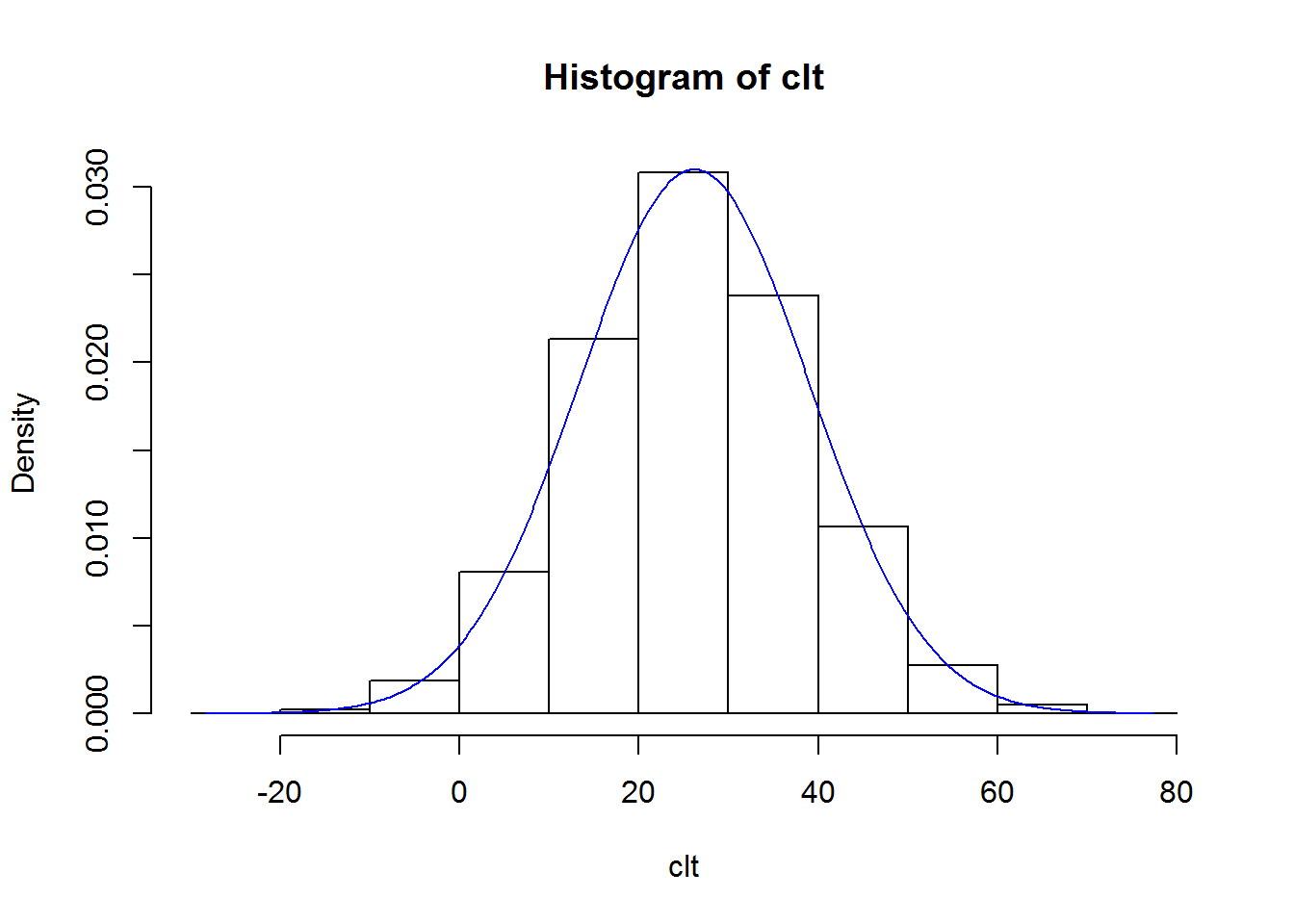rm(X, Y, clt) # Estimating Power and Sample Size ---------------------------------------- # A practical reason to generate data is to estimate statistical power or an # appropriate sample size for an experiment. Power is the probability of # correctly rejecting the null hypothesis when it is actually false. If our # sample is too small, we may fail to reject the null even it truly is false. # EXAMPLE: The two-sample t-test is used to determine if two population means # are equal. The null is the means are the same. An appropriate sample size is # one that is no bigger than it needs to be. An appropriate sample size for such # a test depends on... # - the hypothesized difference between the means (effect size) # - the standard deviation of the populations # - the significance level of our test # - our desired power (usually 0.8) # There is a function in R that allows you to calculate power and sample size # for a t-test: # calculate power for n=20 in each group, SD=1, sig level=0.05 and difference of # means assumed to be 1 (delta): power.t.test(n = 20, delta = 1, sd = 1, sig.level = 0.05) ## ## Two-sample t test power calculation ## ## n = 20 ## delta = 1 ## sd = 1 ## sig.level = 0.05 ## power = 0.8689528 ## alternative = two.sided ## ## NOTE: n is number in *each* group # calculate sample size for power=0.80, SD=1, sig level=0.05 and difference of # means assumed to be 1 (delta): power.t.test(power = 0.80, delta = 1, sd = 1, sig.level = 0.05) ## ## Two-sample t test power calculation ## ## n = 16.71477 ## delta = 1 ## sd = 1 ## sig.level = 0.05 ## power = 0.8 ## alternative = two.sided ## ## NOTE: n is number in *each* group # Always round n to next largest integer # Now let's do a t-test with some simulated data to estimate power via # simulation. Below we simulate 20 observations from two normal distributions, # one with mean 5, and the other with mean 6. We then run a t-test to test the # null hypothesis that both samples come from the same normal distribution # against the alternative hypothesis that they do not. tout <- t.test(rnorm(20,5,1), rnorm(20,6,1)) tout ## ## Welch Two Sample t-test ## ## data: rnorm(20, 5, 1) and rnorm(20, 6, 1) ## t = -3.075, df = 37.903, p-value = 0.003893 ## alternative hypothesis: true difference in means is not equal to 0 ## 95 percent confidence interval: ## -1.5854742 -0.3265825 ## sample estimates: ## mean of x mean of y ## 4.806926 5.762954 # note the structure of tout; it's a list: str(tout) ## List of 9 ##$ statistic  : Named num -3.07
##   ..- attr(*, "names")= chr "t"
##  $parameter : Named num 37.9 ## ..- attr(*, "names")= chr "df" ##$ p.value    : num 0.00389
##  $conf.int : atomic [1:2] -1.585 -0.327 ## ..- attr(*, "conf.level")= num 0.95 ##$ estimate   : Named num [1:2] 4.81 5.76
##   ..- attr(*, "names")= chr [1:2] "mean of x" "mean of y"
##  $null.value : Named num 0 ## ..- attr(*, "names")= chr "difference in means" ##$ alternative: chr "two.sided"
##  $method : chr "Welch Two Sample t-test" ##$ data.name  : chr "rnorm(20, 5, 1) and rnorm(20, 6, 1)"
##  - attr(*, "class")= chr "htest"
# pull out just the p-value
tout$p.value ##  0.003892888 # We can do all that in one shot: t.test(rnorm(20,5,1), rnorm(20,6,1))$p.value
##  0.003047924
# Let's run 1000 such t-tests using the replicate function:
out <- replicate(1000, t.test(rnorm(20,5,1), rnorm(20,6,1))$p.value) # Estimate "power": proportion of times we rejected null of equal means mean(out < 0.05) ##  0.882 # This agrees with the results from power.t.test: power.t.test(n = 20, delta = 1, sd = 1, sig.level = 0.05) ## ## Two-sample t test power calculation ## ## n = 20 ## delta = 1 ## sd = 1 ## sig.level = 0.05 ## power = 0.8689528 ## alternative = two.sided ## ## NOTE: n is number in *each* group # We can also use simulation of two-sample t tests to evaluate various sample # sizes. Let's create a function that simulates data in two groups and outputs # the p-value of a t-test. genTTest <- function(size){ g1 <- rnorm(size,5,1) g2 <- rnorm(size,6,1) t.test(g1,g2)$p.value
}

genTTest(size=10)
##  0.03602279
# Now let's replicate our function 500 times to see how often we get a
# p-value less than 0.05 when n = 10
r.out <- replicate(n = 500, genTTest(size=10))
mean(r.out < 0.05) # our estimate of Power
##  0.58
# We could actually incorporate the above steps into a single function to save
# steps.

# Define a function called tPower that runs N=1000 t-tests and outputs power
# given "size" (sample size in each group), for the genTTest function:
tPower <- function(size){
out <- replicate(1000, genTTest(size))
mean(out < 0.05)
}

# Estimated power with n=10 (10 in each group) for N=1000 t-tests
tPower(size=10)
##  0.547
# Now run the tPower function for increasing levels of sample size (10 - 30)
n <- 10:30
p.est <- sapply(n,tPower) # this may take a moment
plot(n, p.est, type="b", ylab="Power")
abline(h=0.8) # add line for 80% power

# The smallest value of n that saw over 80% of p-values below 0.05
min(n[p.est > 0.8])
##  17
# Again this should agree with what power.t.test() tells us:
power.t.test(delta = 1, sig.level = 0.05, power = 0.80)
##
##      Two-sample t test power calculation
##
##               n = 16.71477
##           delta = 1
##              sd = 1
##       sig.level = 0.05
##           power = 0.8
##     alternative = two.sided
##
## NOTE: n is number in *each* group
# The nice thing about simulation and the R programming language is that we can
# simulate data and results that are not covered by the many assumptions of the
# usual power calculations. power.t.test() assumes equal group sizes and a
# common standard deviation. Using simulation, we can approximate power and
# sample size for a t.test between groups with unequal sample size and different
# standard deviations.

# Let's say we'll sample two groups with a 2:1 ratio and we suspect one group is
# more variable. We'd like to be able to detect a difference as small as 2
# between the two groups.
out <- replicate(1000, t.test(rnorm(20,5,5), rnorm(40,3,2))$p.value) # Estimate "power": proportion of times we rejected null of equal means mean(out < 0.05) ##  0.373 # Not great. What if we set n1=35 and n2=70? mean(replicate(1000, t.test(rnorm(35,5,5), rnorm(70,3,2))$p.value) < 0.05)
##  0.596
# What if we're willing to assume the first group has SD of 3 instead of 5?
mean(replicate(1000, t.test(rnorm(35,5,3), rnorm(70,3,2))$p.value) < 0.05) ##  0.934 # We can pretty much do this for any statistical test or model, it just gets a # little more complicated. # Let's say we intend to collect data on mothers and their babies and we hope to # show that gestation time, age of the mother, and weight of the mother are all # significant contributors to a baby's birth weight (in ounces). Once this data # is collected we might wish to perform a multiple regression where we model a # baby's birth weight as a linear combination (or weighted sum) of gestation # time, age of the mother, and weight of the mother. How many mothers and their # babies do we need to observe? # Note: the idea for this example comes from the "babies" data set included with # the UsingR package. # Let's first simulate some data: n <- 50 gestation <- round(runif(n,240,300)) # gestation time in days age <- round(rnorm(n,26,5)) # age of mother in years mwt <- round(rnorm(n,138,20)) # mother's weight in lbs # These are imperfect simulations. It could result in simulated mother who's 14, # weighs 100, with a gestation time of 300 days. (Unlikely) With more effort and # subject expertise we could probably come up with a more realistic way to # simulate data, but for now this will do. # Now using our mothers, let's simulate birth weights in ounces. These will be # based on a weighted sum of our mothers' data and random noise (ie, factors # that we have not accounted for that contribute to a baby's birth weight.) # That's one of the assumptions of a linear model. # Let's say we want to detect the following if it's true: # - every day of gestation leads to an additional 0.5 ounces of birth weight. # - each additional year of mother's age adds 0.1 ounces to birth weight. # - each additional pound of the mother's weight adds 0.1 ounces to birth weight. # These are the weights in our linear combination, or the coefficients in our # model. We add random noise from a normal distribution with mean 0 and a # standard deviation of 15. This is another assumption of a classic linear # model: the errors are normally distributed with mean 0 and a constant # variance. bwt <- 0.5*gestation + 0.1*age + 0.1*mwt + rnorm(n,0,15) # Now let's regress bwt on gestation, age and mwt. This basically attempts to # recover the parameters we used to simulate the data. summary(lm(bwt ~ gestation + age + mwt)) ## ## Call: ## lm(formula = bwt ~ gestation + age + mwt) ## ## Residuals: ## Min 1Q Median 3Q Max ## -42.166 -9.172 2.075 11.085 28.383 ## ## Coefficients: ## Estimate Std. Error t value Pr(>|t|) ## (Intercept) 32.34873 42.86074 0.755 0.4543 ## gestation 0.32319 0.15331 2.108 0.0405 * ## age 0.79730 0.49156 1.622 0.1116 ## mwt 0.05642 0.11364 0.496 0.6219 ## --- ## Signif. codes: 0 '***' 0.001 '**' 0.01 '*' 0.05 '.' 0.1 ' ' 1 ## ## Residual standard error: 16.12 on 46 degrees of freedom ## Multiple R-squared: 0.1547, Adjusted R-squared: 0.09957 ## F-statistic: 2.806 on 3 and 46 DF, p-value: 0.05004 # The coefficient estimates in the output are the estimated weights in our # linear model. Likewise the residual standard error is the estimated standard # deviation of our error distribution. # Of interest is whether or not the coefficients are deemed significant. They # are definitely part of the data generation process, but how do they stand out # against the noise given various sample sizes? # We can save the summary and extract the coefficient as matrix sout <- summary(lm(bwt ~ gestation + age + mwt)) str(sout) # a list ## List of 11 ##$ call         : language lm(formula = bwt ~ gestation + age + mwt)
##  $terms :Classes 'terms', 'formula' length 3 bwt ~ gestation + age + mwt ## .. ..- attr(*, "variables")= language list(bwt, gestation, age, mwt) ## .. ..- attr(*, "factors")= int [1:4, 1:3] 0 1 0 0 0 0 1 0 0 0 ... ## .. .. ..- attr(*, "dimnames")=List of 2 ## .. .. .. ..$ : chr [1:4] "bwt" "gestation" "age" "mwt"
##   .. .. .. ..$: chr [1:3] "gestation" "age" "mwt" ## .. ..- attr(*, "term.labels")= chr [1:3] "gestation" "age" "mwt" ## .. ..- attr(*, "order")= int [1:3] 1 1 1 ## .. ..- attr(*, "intercept")= int 1 ## .. ..- attr(*, "response")= int 1 ## .. ..- attr(*, ".Environment")=<environment: R_GlobalEnv> ## .. ..- attr(*, "predvars")= language list(bwt, gestation, age, mwt) ## .. ..- attr(*, "dataClasses")= Named chr [1:4] "numeric" "numeric" "numeric" "numeric" ## .. .. ..- attr(*, "names")= chr [1:4] "bwt" "gestation" "age" "mwt" ##$ residuals    : Named num [1:50] 24.92 -22.02 -4.71 11.12 5.08 ...
##   ..- attr(*, "names")= chr [1:50] "1" "2" "3" "4" ...
##  $coefficients : num [1:4, 1:4] 32.3487 0.3232 0.7973 0.0564 42.8607 ... ## ..- attr(*, "dimnames")=List of 2 ## .. ..$ : chr [1:4] "(Intercept)" "gestation" "age" "mwt"
##   .. ..$: chr [1:4] "Estimate" "Std. Error" "t value" "Pr(>|t|)" ##$ aliased      : Named logi [1:4] FALSE FALSE FALSE FALSE
##   ..- attr(*, "names")= chr [1:4] "(Intercept)" "gestation" "age" "mwt"
##  $sigma : num 16.1 ##$ df           : int [1:3] 4 46 4
##  $r.squared : num 0.155 ##$ adj.r.squared: num 0.0996
##  $fstatistic : Named num [1:3] 2.81 3 46 ## ..- attr(*, "names")= chr [1:3] "value" "numdf" "dendf" ##$ cov.unscaled : num [1:4, 1:4] 7.06925 -0.02294 -0.02032 -0.00252 -0.02294 ...
##   ..- attr(*, "dimnames")=List of 2
##   .. ..$: chr [1:4] "(Intercept)" "gestation" "age" "mwt" ## .. ..$ : chr [1:4] "(Intercept)" "gestation" "age" "mwt"
##  - attr(*, "class")= chr "summary.lm"
sout$coefficients ## Estimate Std. Error t value Pr(>|t|) ## (Intercept) 32.34873403 42.8607415 0.7547404 0.45425160 ## gestation 0.32319414 0.1533127 2.1080720 0.04050277 ## age 0.79729874 0.4915647 1.6219611 0.11164676 ## mwt 0.05642102 0.1136416 0.4964822 0.62191855 # Extract the p-values sout$coefficients[-1,4] # p-values
##  gestation        age        mwt
## 0.04050277 0.11164676 0.62191855
# What is less than 0.05?
sout$coefficients[-1,4] < 0.05 ## gestation age mwt ## TRUE FALSE FALSE # We can combine everything into a function and replicate it to see how various # values of n affect the proportion of times we deem a coefficient significant # (ie, statistically different from 0) sim_lm <- function(n){ gestation <- round(runif(n,240,300)) age <- round(rnorm(n,26,5)) mwt <- round(rnorm(n,138,20)) bwt <- 0.5*gestation + 0.1*age + 0.1*mwt + rnorm(n,0,15) summary(lm(bwt ~ gestation + age + mwt))$coefficients[-1,4]
}

# a single simulation
sim_lm(n=30) < 0.05
## gestation       age       mwt
##      TRUE     FALSE     FALSE
# Replicate 1000 times
rout <- replicate(n = 1000, sim_lm(n=30))
# rout is a matrix with 3 rows and 1000 columns that stores the results of our
# 1000 simulations.
dim(rout)
##     3 1000
rout[,1:5]
##                  [,1]      [,2]         [,3]       [,4]        [,5]
## gestation 0.002092624 0.3805592 7.701694e-05 0.01226594 0.004855346
## age       0.049350376 0.7905912 1.311719e-01 0.49201502 0.333483037
## mwt       0.097524566 0.9164997 2.424897e-02 0.40210353 0.791018032
# proportion of times predictor declared significant:
apply(rout,1,function(x)mean(x < 0.05))
## gestation       age       mwt
##     0.822     0.050     0.112
# With n = 30, we're definitely detecting the gestation effect, but not so much
# the age and weight.

# What about n = 100?
rout <- replicate(n = 1000, sim_lm(n=100))
apply(rout,1,function(x)mean(x < 0.05))
## gestation       age       mwt
##     1.000     0.052     0.280
# What about n = 1000?
rout <- replicate(n = 1000, sim_lm(n=1000))
apply(rout,1,function(x)mean(x < 0.05))
## gestation       age       mwt
##     1.000     0.183     0.990
# Beware: everything becomes significant if your sample size is made large
# enough since standard error and hence p-values are functions of sample size.

# What we might want to do is standardize the predictor variables so they're all
# on the same scale:
sim_lm <- function(n){
gestation <- round(runif(n,240,300))
age <- round(rnorm(n,26,5))
mwt <- round(rnorm(n,138,20))
bwt <- 0.5*gestation + 0.1*age + 0.1*mwt + rnorm(n,0,5)
summary(lm(bwt ~ scale(gestation) + scale(age) + scale(mwt)))$coefficients[-1,4] } rout <- replicate(n = 1000, sim_lm(n=100)) apply(rout,1,function(x)mean(x < 0.05)) ## scale(gestation) scale(age) scale(mwt) ## 1.000 0.159 0.968 # Generating multi-level data --------------------------------------------- # Let's say we're interested in the effect of a new curriculum on the # improvement of a particular standardized test. We would probably select some # schools, then select teachers in the school, and then randomly assign to each # teacher a new curriculum to use (versus the standard approach). We would # pre-test the students, apply the two curriculums, and then test again to # measure their improvement. In this design, we have teachers nested in schools, # and students nested in teachers. Hence, the name multi-level. It's probable # some schools and teachers are better than others, and hence may contribute to # improvement in test scores. We'd like to control for that variability as well # as measure the effect of curriculum. A multi-level model (or linear mixed # effect model) allows you to do this. # It may be of interest to generate some data to better understand how we might # want to analyze it. This means we'll want a data set with one record per # student, per teacher, per school. # First let's generate school, teacher and student ids # 10 schools school <- 1:10 # generate number of teachers at each school set.seed(1) teacher <- rbinom(n = 10, size = 6, prob = 0.8) teacher # 5 teachers at school 1, 5 teachers at school 2... ##  5 5 5 3 6 4 3 4 5 6 sum(teacher) # 46 teachers ##  46 # generate number of students in each class set.seed(1) student <- rbinom(n = sum(teacher), size = 25, prob = 0.8) length(student) # 46; matches number of teachers ##  46 sum(student) # 911 students ##  911 school; teacher; student ##  1 ##  5 ##  21 # school 1 has 5 teachers; teacher 1 at school 1 has 21 students. # generate school ids; one value for each student; a little tricky! First # generate sid for each teacher, then generate sid for each student. sid <- rep(school, times = teacher) # length = 46 sid <- rep(sid, times = student) # length = 911 length(sid) # 911 ##  911 # generate teacher ids; teachers nested in schools. school; teacher ##  1 ##  5 # For example generate teacher IDs at school 1: seq(teacher) ##  1 2 3 4 5 # apply seq function to each value in the teacher vector: tid <- unlist(sapply(teacher, seq)) length(tid) # 46 ##  46 tid ##  1 2 3 4 5 1 2 3 4 5 1 2 3 4 5 1 2 3 1 2 3 4 5 6 1 2 3 4 1 2 3 1 2 3 4 ##  1 2 3 4 5 1 2 3 4 5 6 # now repeat these ids as many times as each teacher has students tid <- rep(tid, student) length(tid) # 911 ##  911 # now generate stundent ids, students nested in teachers. Don't really need # these, but we'll generate these anyway. student # teacher 1 at school 1 has 21 students ##  21 student # teacher 2 at school 1 has 21 students ##  21 stid <- unlist(sapply(student, seq)) length(stid) ##  911 # let's put in a data frame and see what we got: sDat <- data.frame(sid = factor(sid), tid = factor(tid), stid = factor(stid)) head(sDat, n=25) ## sid tid stid ## 1 1 1 1 ## 2 1 1 2 ## 3 1 1 3 ## 4 1 1 4 ## 5 1 1 5 ## 6 1 1 6 ## 7 1 1 7 ## 8 1 1 8 ## 9 1 1 9 ## 10 1 1 10 ## 11 1 1 11 ## 12 1 1 12 ## 13 1 1 13 ## 14 1 1 14 ## 15 1 1 15 ## 16 1 1 16 ## 17 1 1 17 ## 18 1 1 18 ## 19 1 1 19 ## 20 1 1 20 ## 21 1 1 21 ## 22 1 2 1 ## 23 1 2 2 ## 24 1 2 3 ## 25 1 2 4 # assign treatments; randomly select teachers set.seed(1) trt <- sample(0:1, size = sum(teacher), replace = TRUE) # need to repeat treatment for each teacher at student length sDat$trt <- rep(trt, student)
length(sDat$trt) # 911 ##  911 table(sDat$trt)
##
##   0   1
## 450 461
# Now let's generate a change in score that is better for students with
# treatment 1. Let's say the new - old score is about 15 points better for
# trt==1, while the new - old score is about 10 points better for trt==0.

# Tempting to use ifelse like this, but that doesn't do what you
# want!
# ifelse(sDat$trt==1, rnorm(1, 15, 5), rnorm(1, 10, 5)) # To use ifelse() you have to define the yes and no arguments to be the same # length as the condition. length(sDat$trt==1) == 911
##  TRUE
# So our yes/no arguments need to be length 911 as well.
set.seed(1)
sDat$diff <- ifelse(sDat$trt==1,
rnorm(nrow(sDat), 15, 5),
rnorm(nrow(sDat), 10, 5))

head(sDat, n = 20)
##    sid tid stid trt      diff
## 1    1   1    1   0  7.685903
## 2    1   1    2   0  2.655589
## 3    1   1    3   0 10.763433
## 4    1   1    4   0 18.868813
## 5    1   1    5   0  6.759645
## 6    1   1    6   0  9.000913
## 7    1   1    7   0 13.446219
## 8    1   1    8   0 10.180728
## 9    1   1    9   0 19.717682
## 10   1   1   10   0 13.686069
## 11   1   1   11   0 21.606670
## 12   1   1   12   0 11.744547
## 13   1   1   13   0  4.330417
## 14   1   1   14   0 12.106676
## 15   1   1   15   0  5.377219
## 16   1   1   16   0  4.964688
## 17   1   1   17   0  9.052628
## 18   1   1   18   0 14.669584
## 19   1   1   19   0 11.719550
## 20   1   1   20   0 14.070101
aggregate(diff ~ trt, data=sDat, mean)
##   trt     diff
## 1   0 10.14360
## 2   1 15.06944
aggregate(diff ~ sid + trt, data=sDat, mean)
##    sid trt      diff
## 1    1   0 10.360249
## 2    2   0 10.726033
## 3    3   0  9.866388
## 4    4   0 11.968725
## 5    5   0  9.895554
## 6    6   0 10.517274
## 7    7   0 10.414030
## 8    8   0  9.956987
## 9    9   0  8.623131
## 10   1   1 15.786227
## 11   2   1 14.684297
## 12   3   1 15.532909
## 13   4   1 15.630672
## 14   5   1 14.965114
## 15   7   1 16.072723
## 16   8   1 14.191440
## 17   9   1 15.151115
## 18  10   1 14.901176
# Right now the only source of variation is due to treatment. What if we want to
# incorporate variation due to teacher or school? Let's think of deriving diff
# as a formula:

# diff = 10 + trt*5 + error

# The error can be due to student, teacher and/or school.

# school error (10 schools)
set.seed(1)
s_err <- rnorm(10, 0, 2)
s_err <- rep(s_err, times = teacher) # length = 46
s_err <- rep(s_err, times = student) # length = 911

# teacher error (46 teachers)
set.seed(1)
t_err <- rnorm(46, 0, 3)
t_err <- rep(t_err, times = student)

# student error (911 students)
set.seed(1)
st_err <- rnorm(911, 0, 2)

# derive diff (save as diff2 so we keep our original diff)
sDat$diff2 <- 10 + 5*sDat$trt + s_err + t_err + st_err

head(sDat, n = 20)
##    sid tid stid trt      diff     diff2
## 1    1   1    1   0  7.685903  5.614823
## 2    1   1    2   0  2.655589  7.235018
## 3    1   1    3   0 10.763433  5.196474
## 4    1   1    4   0 18.868813 10.058293
## 5    1   1    5   0  6.759645  7.526746
## 6    1   1    6   0  9.000913  5.226794
## 7    1   1    7   0 13.446219  7.842589
## 8    1   1    8   0 10.180728  8.344380
## 9    1   1    9   0 19.717682  8.019294
## 10   1   1   10   0 13.686069  6.256954
## 11   1   1   11   0 21.606670  9.891293
## 12   1   1   12   0 11.744547  7.647417
## 13   1   1   13   0  4.330417  5.625250
## 14   1   1   14   0 12.106676  2.438331
## 15   1   1   15   0  5.377219  9.117593
## 16   1   1   16   0  4.964688  6.777864
## 17   1   1   17   0  9.052628  6.835350
## 18   1   1   18   0 14.669584  8.755403
## 19   1   1   19   0 11.719550  8.510173
## 20   1   1   20   0 14.070101  8.055534
aggregate(diff2 ~ trt, data=sDat, mean)
##   trt     diff2
## 1   0  9.835481
## 2   1 15.579603
aggregate(diff2 ~ sid + tid + trt, data=sDat, mean)
##    sid tid trt     diff2
## 1    1   1   0  7.318155
## 2    3   1   0 13.333096
## 3    4   1   0 13.396776
## 4    5   1   0 12.641224
## 5    6   1   0 10.447262
## 6    1   2   0  9.158375
## 7    3   2   0  8.900876
## 8    6   2   0  7.829293
## 9    7   2   0 12.082756
## 10   8   2   0 11.740452
## 11   6   3   0  8.114703
## 12   7   3   0 14.983388
## 13   8   3   0 10.896663
## 14   9   3   0 10.892733
## 15   3   4   0  1.788597
## 16   5   4   0 12.777155
## 17   6   4   0  3.611208
## 18   1   5   0  9.843536
## 19   2   5   0  9.531945
## 20   9   5   0 13.473973
## 21   5   6   0  4.455889
## 22   2   1   1 13.209420
## 23   7   1   1 14.969497
## 24   8   1   1 15.952162
## 25   9   1   1 14.860967
## 26  10   1   1 13.507430
## 27   2   2   1 16.717347
## 28   4   2   1 18.485662
## 29   5   2   1 17.561854
## 30   9   2   1 14.920258
## 31  10   2   1 13.733012
## 32   1   3   1 11.795826
## 33   2   3   1 16.587959
## 34   3   3   1 11.561346
## 35   4   3   1 21.158563
## 36   5   3   1 18.689651
## 37  10   3   1 16.904876
## 38   1   4   1 18.563744
## 39   2   4   1 17.439219
## 40   8   4   1 11.902825
## 41   9   4   1 19.721294
## 42  10   4   1 15.681343
## 43   3   5   1 17.033537
## 44   5   5   1 15.476289
## 45  10   5   1 12.761625
## 46  10   6   1 11.804840
# Fit a mixed-effect model with trt as the fixed effect and three sources of
# variation: school, teacher within school, and student
library(lme4)
## Loading required package: Matrix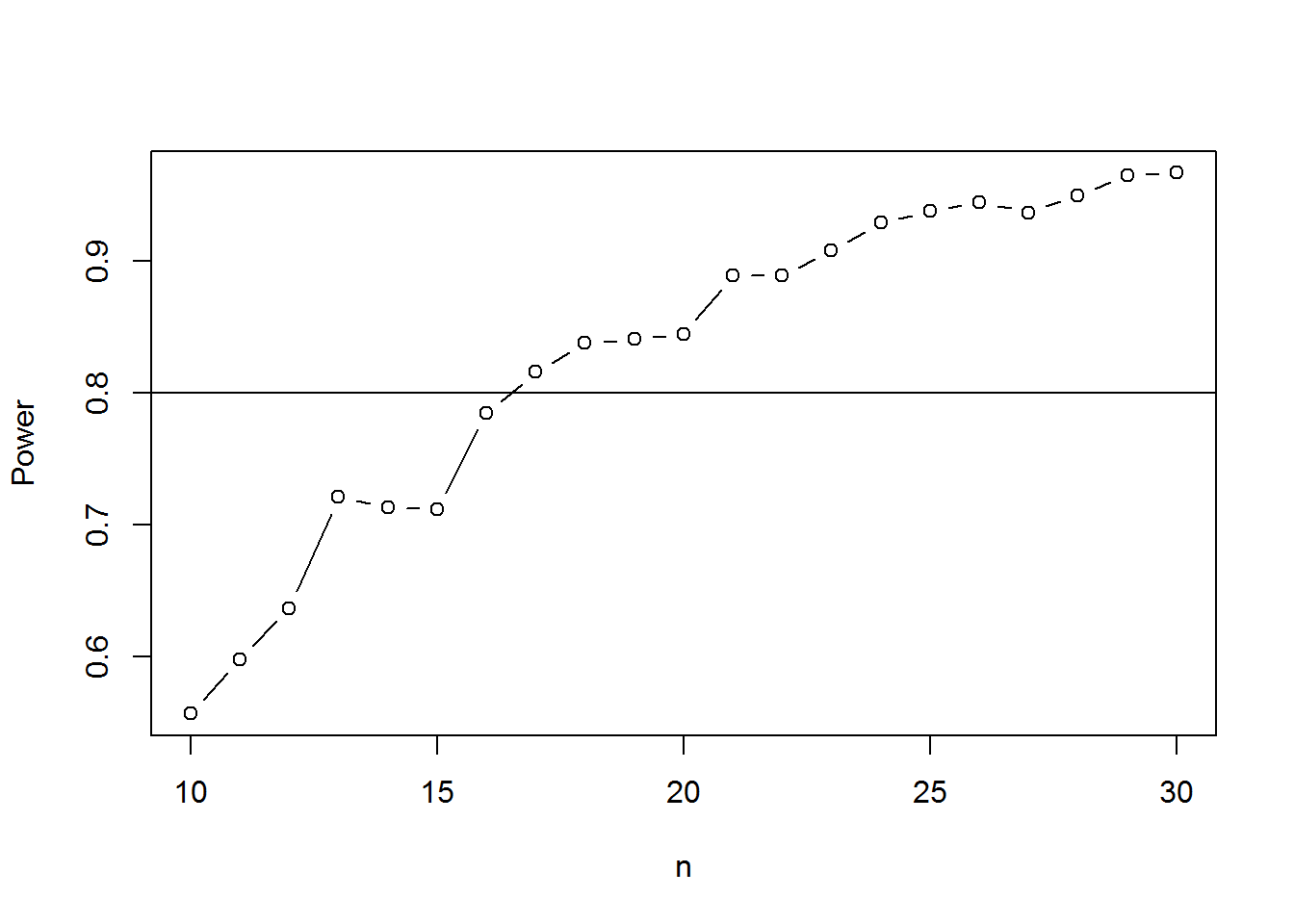lme1 <- lmer(diff2 ~ trt + (1 | sid/tid), data=sDat)
summary(lme1, corr = FALSE)
## Linear mixed model fit by REML ['lmerMod']
## Formula: diff2 ~ trt + (1 | sid/tid)
##    Data: sDat
##
## REML criterion at convergence: 4088.5
##
## Scaled residuals:
##     Min      1Q  Median      3Q     Max
## -2.7028 -0.6533 -0.0036  0.6830  3.8205
##
## Random effects:
##  Groups   Name        Variance Std.Dev.
##  tid:sid  (Intercept) 7.864    2.804
##  sid      (Intercept) 1.419    1.191
##  Residual             4.333    2.082
## Number of obs: 911, groups:  tid:sid, 46; sid, 10
##
## Fixed effects:
##             Estimate Std. Error t value
## (Intercept)   9.9952     0.7425  13.461
## trt           5.6743     0.9011   6.297
# Again, this basically attempts to recover the parameters we used to simulate
# the data.

# Bootstrapping/Resampling ------------------------------------------------

# Bootstrapping means treating our sample like a surrogate population,
# resampling from it many times over, and calculating a statistic of interest
# each time. We can then use these simulated statistics to make some inferences
# about the uncertainty of our original estimate.

# EXAMPLE 1: estimate the median Temperation in Charlottesville in February.
hist(weather$Mean.TemperatureF[weather$Month=="Feb"])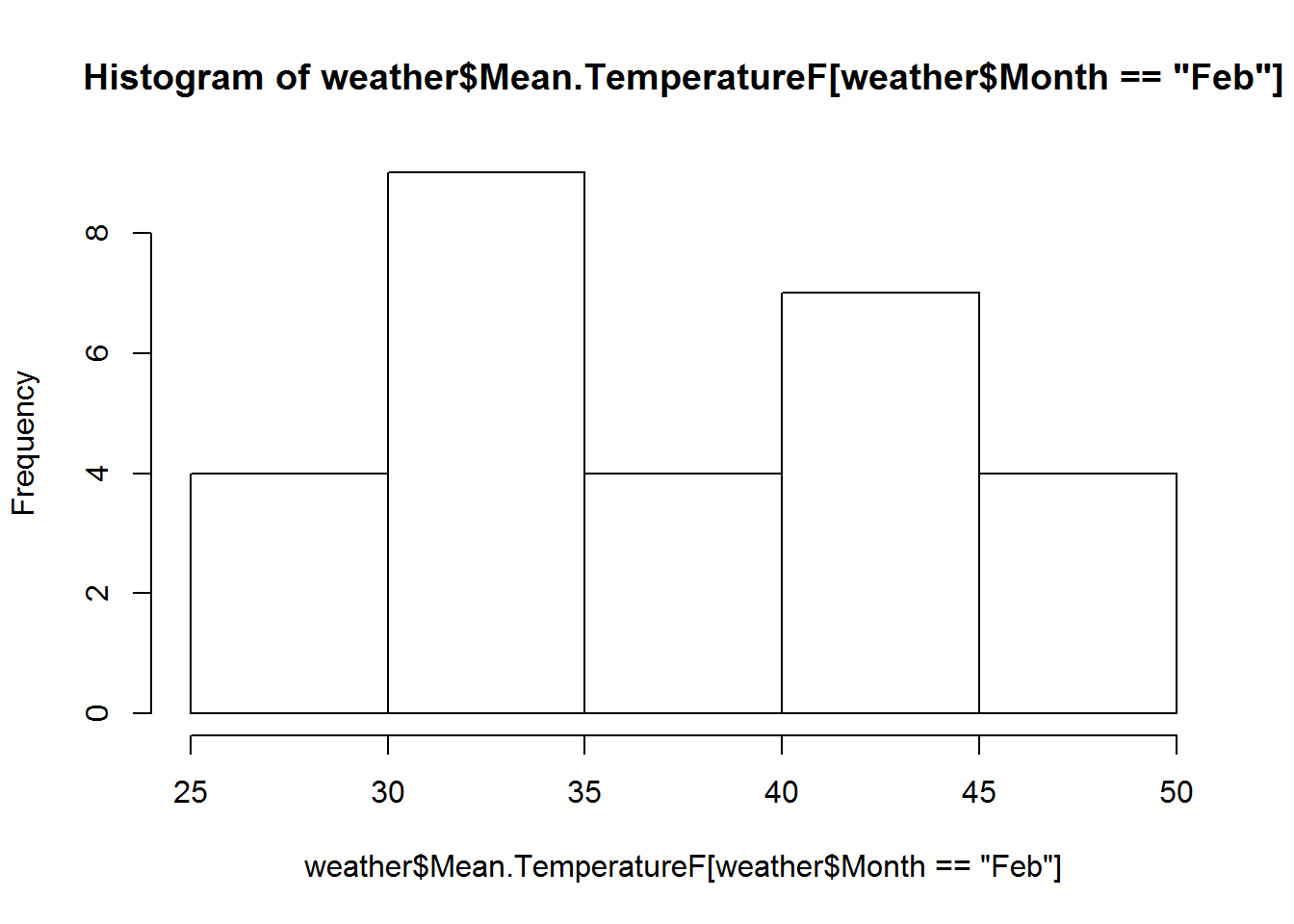temps <- weather$Mean.TemperatureF[weather$Month=="Feb"]
median(temps)
##  37
# The standard error gives some indication of how uncertain my estimate is.
# There is a formula for the standard error of the median, but it can be wrong
# for extremely non-normal distributions. Let's use the bootstrap instead.

# The basic idea is to resample my data with replacement, calculate the median,
# store it, and repeat many times, usually about 1000.

# Doing it once
median(sample(temps, replace = TRUE))
##  36
# Doing it 999 times and storing
bmed <- replicate(n = 999, median(sample(temps, replace = TRUE)))

# Calculating the standard deviation of our bootstrapped medians provides an
# estimate of the standard error.
sd(bmed)
##  2.172237
hist(bmed)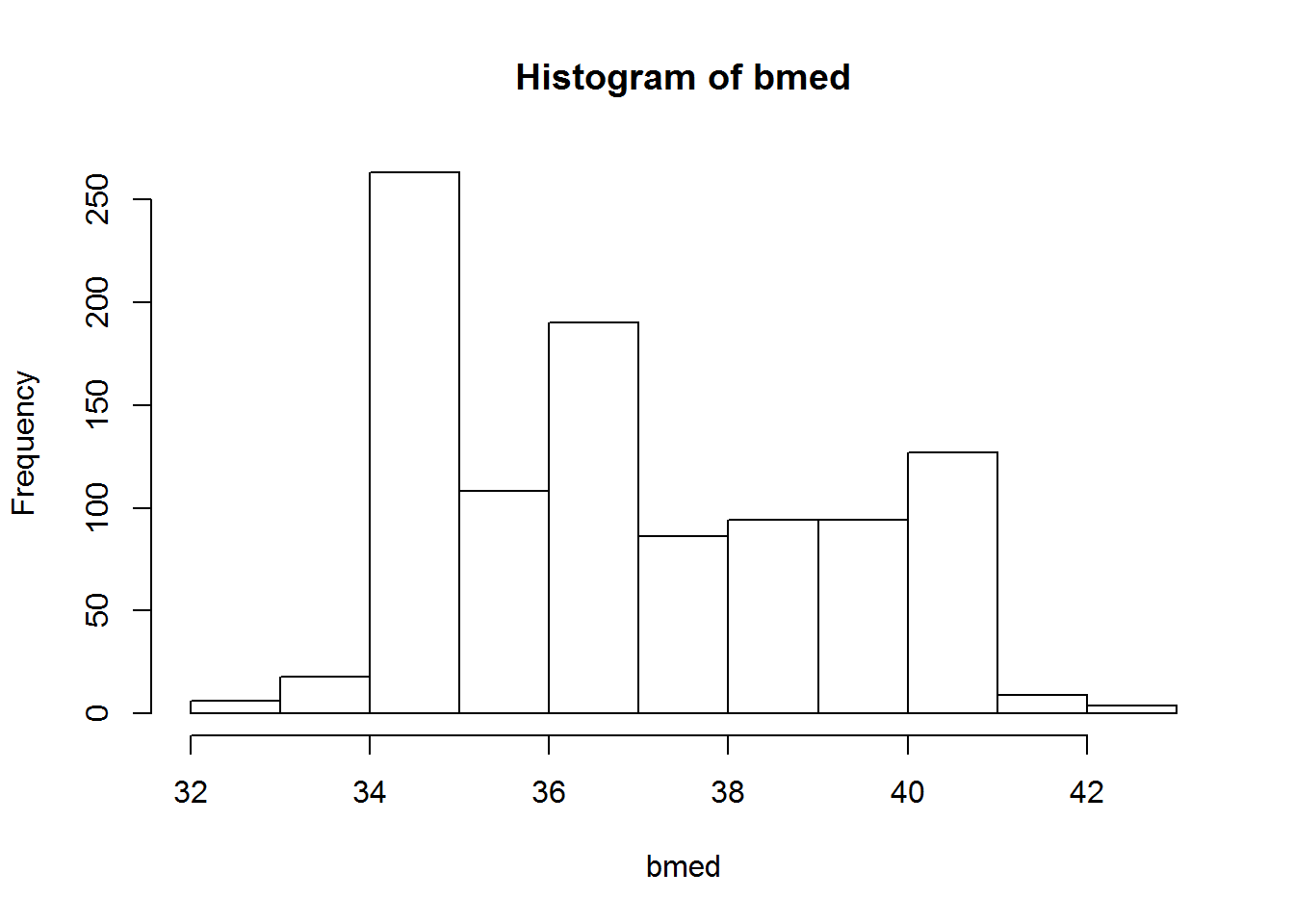# The histogram of my bootstrapped medians is not symmetric, so I may prefer a
# percentile confidence interval instead of the usual "add/substract 2 SEs to my
# estimate".
quantile(bmed, probs = c(0.025, 0.975))
##  2.5% 97.5%
##  34.5  41.0
# EXAMPLE 2: correlation of Temperature and Dew Point
plot(MeanDew.PointF ~ Mean.TemperatureF, data=weather)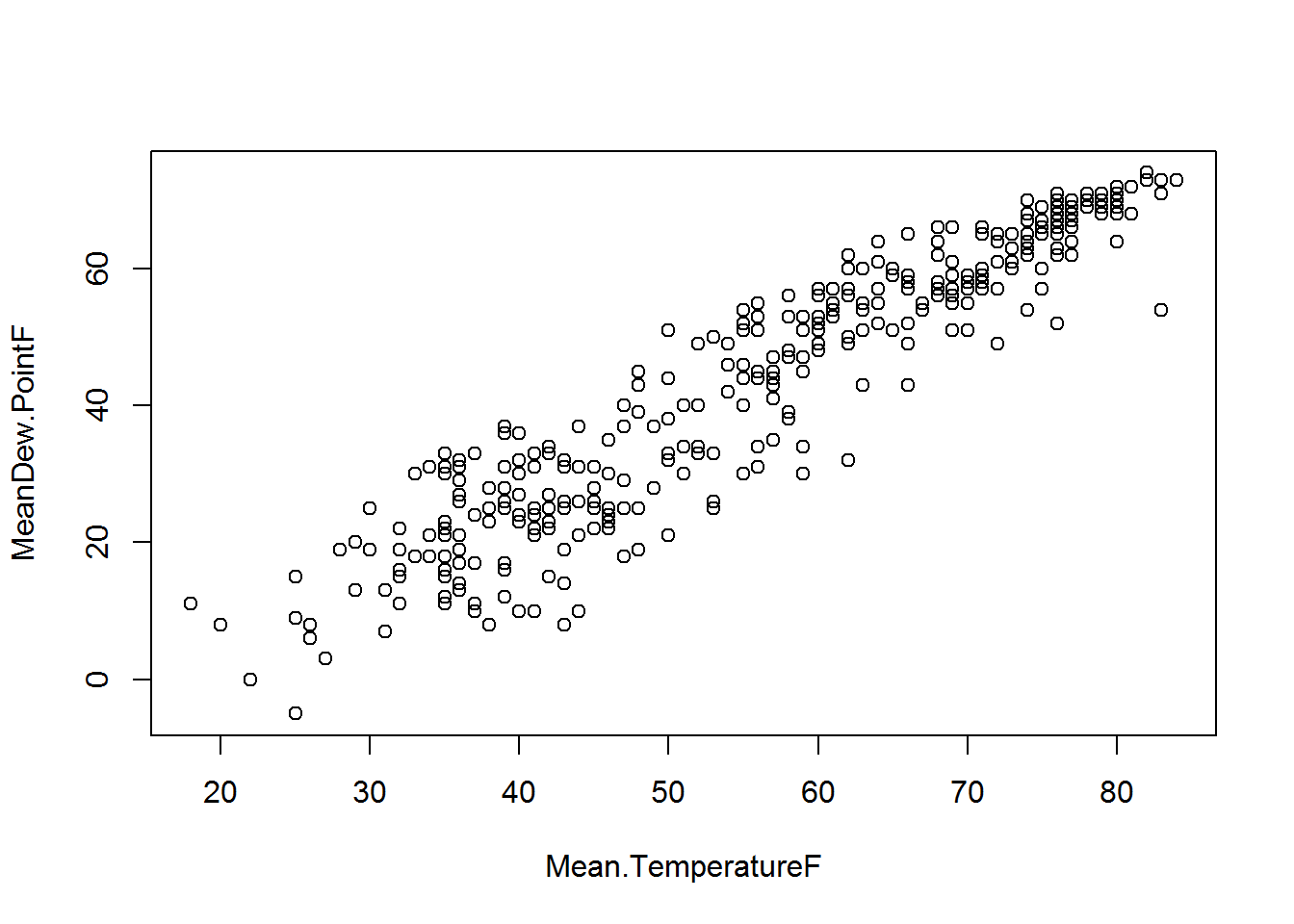with(weather, cor(Mean.TemperatureF, MeanDew.PointF, use = "complete.obs"))
##  0.9410504
# Let's use the bootstrap to estimate the standard error of the correlation:

# First we subset our data:
corrData <- weather[,c("Mean.TemperatureF", "MeanDew.PointF")]

# write function to resample data and calculate correlation. Notice you can
# write a function without arguments.
cor.fun <- function(){
k <- sample(nrow(corrData), replace = TRUE)
cor(corrData[k,1], corrData[k,2], use = "complete.obs")
}

# Try it out one time
cor.fun()
##  0.9386767
# Replicate the function 999 times and save
bcorr <- replicate(n = 999, cor.fun())
sd(bcorr) # estimate of standard error
##  0.005520638
hist(bcorr)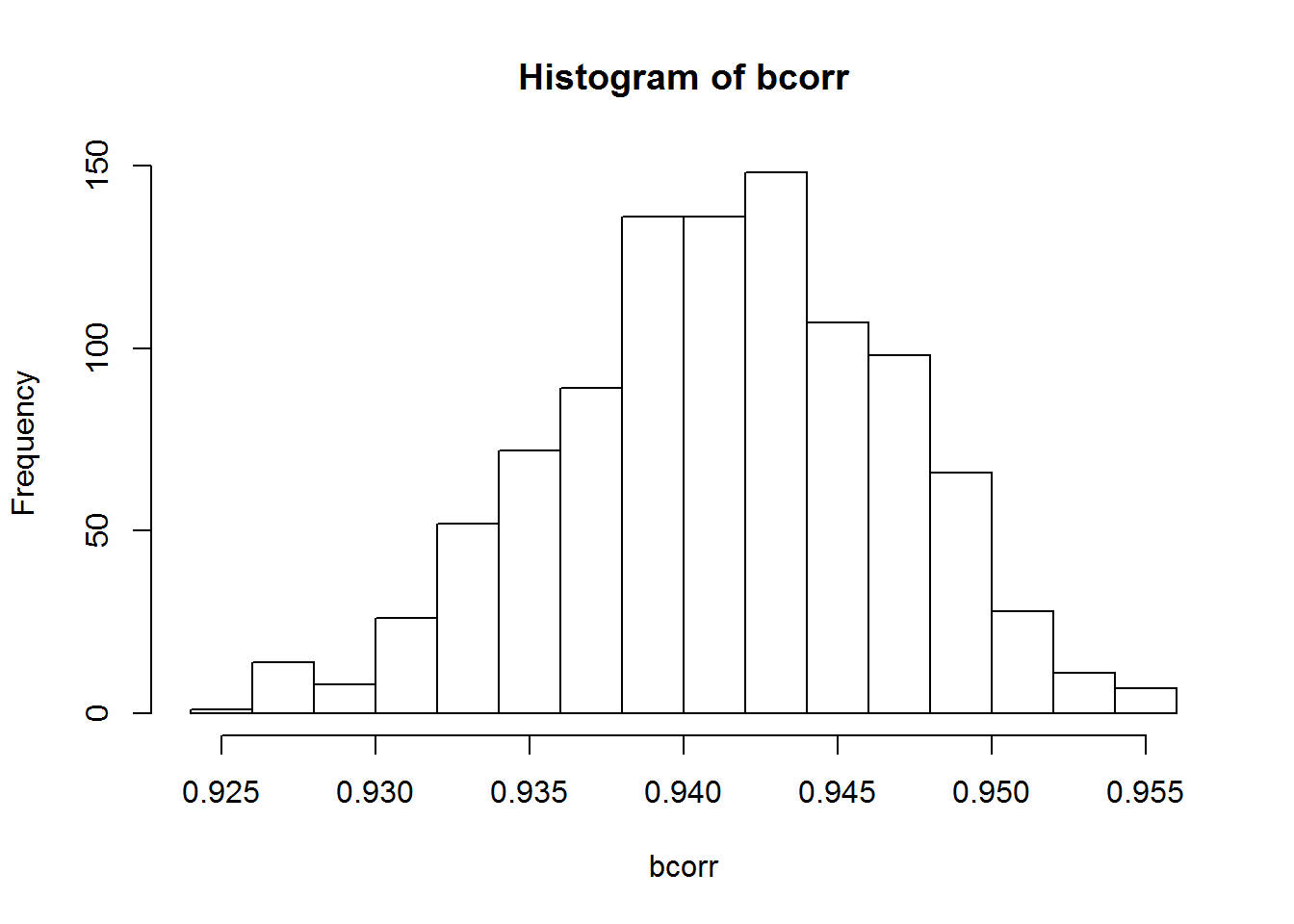# The distribution is pretty symmetric so we could add/substract the SE to form a confidence interval:
cor.est <- with(weather, cor(Mean.TemperatureF, MeanDew.PointF, use = "complete.obs"))
cor.est + c(-2*sd(bcorr), 2*sd(bcorr))
##  0.9300091 0.9520917
# Almost identical to the percentile confidence interval
quantile(bcorr, probs = c(0.025, 0.975))
##      2.5%     97.5%
## 0.9301909 0.9514701
# For more information on Resampling Methods, see a previous workshop of mine:
# http://static.lib.virginia.edu/statlab/materials/workshops/ResamplingMethods.zip

# Time-permitting bonus material ------------------------------------------

# The wakefield package ---------------------------------------------------

# The wakefield package provides functions for generating random data sets.

# install.packages("wakefield")
library(wakefield)
##
## Attaching package: 'wakefield'
## The following object is masked from 'package:lme4':
##
##     dummy
# Let's look at a few of the functions.

# Generate Random Vector of animals
animal(n = 20) # samples 20 of 10 different animals from wakefield's "animal_list"
##   Tiger Shark            Minke Whale            Indri
##   Minke Whale            Keel Billed Toucan     Keel Billed Toucan
##   American Water Spaniel Keel Billed Toucan     Puss Moth
##  Leopard                Malayan Civet          American Water Spaniel
##  Malayan Civet          Tiger Shark            Malayan Civet
##  Woodlouse              Puss Moth              Minke Whale
##  Puss Moth              Walrus
## 10 Levels: Walrus Minke Whale Woodlouse Keel Billed Toucan ... Indri
animal(n = 20, k=20) # 20 levels
##   Monte Iberia Eleuth     Bichon Frise
##   Frigatebird             Monte Iberia Eleuth
##   Numbat                  Leaf-Tailed Gecko
##   Fur Seal                Hyena
##   Spectacled Bear         Hippopotamus
##  Magpie                  Bichon Frise
##  Spectacled Bear         Fur Seal
##  Butterfly Fish          Bichon Frise
##  Quetzal                 Frigatebird
##  Western Lowland Gorilla Numbat
## 20 Levels: Blue Whale Grey Seal Macaroni Penguin ... Badger
# pets
# pet(n, x = c("Dog", "Cat", "None", "Bird", "Horse"),
#     prob = c(0.365, 0.304, 0.258, 0.031, 0.015), name = "Pet")
pet(n = 15)
##   Cat  Dog  None Cat  None Bird Dog  None None Cat  Cat  Dog  Dog  Cat
##  Cat
## Levels: Dog Cat None Bird Horse
pet(n = 15, x = c("Dog", "Cat", "Bird", "Lizard"),
prob = c(0.4,0.3,0.2,0.1))
##   Dog    Dog    Dog    Dog    Dog    Dog    Dog    Cat    Bird   Bird
##  Cat    Dog    Cat    Dog    Lizard
## Levels: Dog Cat Bird Lizard
# Generate Random Vector of Educational Attainment Level
education(n = 15)
##   Regular High School Diploma
##   Regular High School Diploma
##   Bachelor's Degree
##   Bachelor's Degree
##   GED or Alternative Credential
##   Some College, 1 or More Years, No Degree
##   Some College, Less than 1 Year
##   Regular High School Diploma
##   Bachelor's Degree
##  GED or Alternative Credential
##  Regular High School Diploma
##  Associate's Degree
##  Master's Degree
##  Regular High School Diploma
##  Regular High School Diploma
## 12 Levels: No Schooling Completed ... Doctorate Degree
# The educational attainments and probabilities used match approximate U.S.
# educational attainment make-up

# Generate Random Vector of Control/Treatment Groups
group(10)
##   Control   Treatment Treatment Treatment Treatment Control   Treatment
##   Treatment Treatment Control
## Levels: Control Treatment
# Using the r_data_frame() function allows you to create a data frame of random
# values. From the r_data_frame examples:

r_data_frame(n = 30,
id,             # these are all functions
race,
age,
sex,
hour,
iq,
height,
died,
Scoring = rnorm, # random normal data N(0,1)
Smoker = valid   # Random Logical Vector using valid function
)
## Source: local data frame [30 x 10]
##
##       ID   Race   Age    Sex     Hour    IQ Height  Died     Scoring
##    (chr) (fctr) (int) (fctr)   (tims) (dbl)  (dbl) (lgl)       (dbl)
## 1     01  White    24 Female 00:30:00   105     72  TRUE -0.42384178
## 2     02  White    28 Female 01:30:00   116     73  TRUE  0.78933460
## 3     03  White    27 Female 02:30:00    95     67 FALSE -0.01383229
## 4     04  White    32   Male 04:00:00    96     68 FALSE -0.79367513
## 5     05  White    30   Male 04:30:00   101     64  TRUE  0.56633073
## 6     06  White    25 Female 04:30:00    81     67  TRUE  0.03708203
## 7     07  White    33 Female 05:00:00   128     68  TRUE  0.25376948
## 8     08  White    20   Male 05:00:00    91     69  TRUE -0.81342610
## 9     09  White    32   Male 06:30:00    97     68  TRUE  0.39539876
## 10    10  Black    20 Female 07:00:00    89     70  TRUE -2.06715442
## ..   ...    ...   ...    ...      ...   ...    ...   ...         ...
## Variables not shown: Smoker (lgl)
# Notice it returs a dplyr tbl_df.

rData <- r_data_frame(n = 30,
id,
race,
age(x = 8:14),
Gender = sex,
Time = hour,
iq,
grade, grade, grade,  #repeated measures
height(mean=50, sd = 10),
died,
Scoring = rnorm,
Smoker = valid)

aggregate(Grade_1 ~ Gender, data=rData, mean)
##   Gender  Grade_1
## 1   Male 88.11875
## 2 Female 88.27143
# generate dummy coded variables
r_data_frame(n=100,
id,
age,
r_dummy(sex, prefix=TRUE),
r_dummy(political)
)
## Source: local data frame [100 x 7]
##
##       ID   Age Sex_Male Sex_Female Constitution Democrat Libertarian
##    (chr) (int)    (int)      (int)        (int)    (int)       (int)
## 1    001    29        1          0            0        1           0
## 2    002    25        0          1            0        1           0
## 3    003    20        0          1            1        0           0
## 4    004    33        1          0            1        0           0
## 5    005    21        0          1            1        0           0
## 6    006    28        1          0            1        0           0
## 7    007    25        1          0            0        1           0
## 8    008    29        1          0            0        1           0
## 9    009    23        0          1            1        0           0
## 10   010    29        1          0            1        0           0
## ..   ...   ...      ...        ...          ...      ...         ...
# Friendly vignette is available here:
# https://github.com/trinker/wakefield/blob/master/README.md

# A demonstration of familywise error rate --------------------------------

# In statistics, familywise error rate (FWER) is the probability of making one
# or more false discoveries, or type I errors, among all the hypotheses when
# performing multiple hypotheses tests.

n <- 1000; p <- 20 # 1000 observations, 20 variables

# generate all data from N(0,1)
set.seed(5)
dat <- as.data.frame(matrix(rnorm(n*p),ncol = p))
head(dat)
##            V1           V2         V3         V4          V5          V6
## 1 -0.84085548 -1.455054580  1.5540221  0.4743471  0.39961801 -1.07791094
## 2  1.38435934  1.244562895 -0.6617420  0.4016796 -0.08949395  0.03900705
## 3 -1.25549186 -0.431947066  0.1040932 -0.8148507  0.82074128 -1.16841845
## 4  0.07014277  0.006869276 -0.8564106 -0.5137524  1.52455245 -0.88609118
## 5  1.71144087  0.124551049 -1.3303153 -0.1382446 -0.06820091 -1.11318729
## 6 -0.60290798 -0.409628630 -1.5975543  1.1694695  0.73546212  0.79146280
##           V7         V8         V9         V10         V11        V12
## 1 -0.8803930  0.8109258 -0.9019425  0.06133178 -0.42076660  1.3209526
## 2  0.7782903  0.9880565  0.3025380  0.95665412  0.04973967 -1.7163007
## 3  0.7686086  0.2754796  0.7604483 -0.78828213  0.10078859  2.0624219
## 4 -1.1541475  0.5016297 -0.4935995  0.37548753  0.65645650  0.5401959
## 5 -1.4466186 -0.9135625 -1.2956919  1.28862804 -0.28103800 -0.3296635
## 6 -1.2292040  0.5633001  0.7070963  2.07726075  0.67818179  0.6883394
##          V13        V14         V15        V16         V17        V18
## 1  0.2428015  0.8644350  0.92058566 -0.1211701 -0.86003688  0.8238042
## 2 -1.6830241  1.4599678  0.79684624 -1.0613289  1.06794159  0.8945495
## 3 -0.9657010 -1.6561345 -0.82773341 -1.2304916  0.45460781 -1.4416087
## 4  1.6341124 -0.8139118 -0.01256819  0.2167260 -0.03416298 -2.1548747
## 5  0.7682981 -2.5148183  1.41860448 -1.5168992  0.72160150 -0.9165946
## 6 -1.0877496  1.3888039  0.49498683  2.1691646 -1.21656524 -0.2938999
##          V19         V20
## 1 -0.2647265  0.28246610
## 2 -0.5083911 -1.46066056
## 3 -0.9235738  0.81242679
## 4 -0.6683567 -0.18111757
## 5 -0.5796659  0.42584388
## 6 -0.3445819  0.07244771
# Let's say V1 is our response (or dependent variable) and V2 - V20 are
# predictors (or independent variables). Let's do multiple regression and
# regress V1 on V2 - V20. None of these predictors "explain" the variability in
# V1. All data is random normal N(0,1). Yet we will likely see some predictors
# declared as significant.

# lm(V1 ~ ., data=dat) regresses V1 on all other columns in dat. summary()
# returns the coefficients and associated t-tests for significance.

m1 <- lm(V1 ~ ., data=dat)
summary(m1)
##
## Call:
## lm(formula = V1 ~ ., data = dat)
##
## Residuals:
##     Min      1Q  Median      3Q     Max
## -3.2463 -0.6647  0.0168  0.6618  3.3408
##
## Coefficients:
##               Estimate Std. Error t value Pr(>|t|)
## (Intercept)  0.0168370  0.0324992   0.518   0.6045
## V2           0.0163880  0.0326681   0.502   0.6160
## V3           0.0030458  0.0314732   0.097   0.9229
## V4           0.0252702  0.0330391   0.765   0.4445
## V5           0.0014738  0.0319308   0.046   0.9632
## V6           0.0108766  0.0321140   0.339   0.7349
## V7           0.0658600  0.0331993   1.984   0.0476 *
## V8           0.0288425  0.0312278   0.924   0.3559
## V9           0.0327566  0.0327270   1.001   0.3171
## V10          0.0185037  0.0309837   0.597   0.5505
## V11          0.0024442  0.0326863   0.075   0.9404
## V12         -0.0163250  0.0327013  -0.499   0.6177
## V13         -0.0436959  0.0316654  -1.380   0.1679
## V14         -0.0099237  0.0324260  -0.306   0.7596
## V15          0.0038890  0.0324829   0.120   0.9047
## V16         -0.0100565  0.0323146  -0.311   0.7557
## V17         -0.0304926  0.0313994  -0.971   0.3317
## V18          0.0002955  0.0312967   0.009   0.9925
## V19         -0.0254945  0.0325917  -0.782   0.4343
## V20          0.0119872  0.0318887   0.376   0.7071
## ---
## Signif. codes:  0 '***' 0.001 '**' 0.01 '*' 0.05 '.' 0.1 ' ' 1
##
## Residual standard error: 1.016 on 980 degrees of freedom
## Multiple R-squared:  0.01189,    Adjusted R-squared:  -0.007267
## F-statistic: 0.6207 on 19 and 980 DF,  p-value: 0.893
# V7 appears to be significant, but in fact is not.

# we can the summary results
sout <- summary(m1)
typeof(sout)
##  "list"
# str(sout)
sout$coefficients ## Estimate Std. Error t value Pr(>|t|) ## (Intercept) 0.0168370374 0.03249916 0.518076002 0.60452218 ## V2 0.0163879554 0.03266808 0.501650408 0.61602613 ## V3 0.0030457813 0.03147321 0.096773787 0.92292583 ## V4 0.0252701688 0.03303907 0.764857250 0.44454069 ## V5 0.0014737767 0.03193076 0.046155394 0.96319580 ## V6 0.0108765647 0.03211397 0.338686351 0.73491858 ## V7 0.0658599961 0.03319935 1.983773688 0.04755975 ## V8 0.0288424581 0.03122780 0.923614753 0.35591428 ## V9 0.0327566168 0.03272699 1.000905134 0.31711974 ## V10 0.0185036810 0.03098369 0.597207123 0.55050706 ## V11 0.0024442469 0.03268632 0.074778891 0.94040590 ## V12 -0.0163249541 0.03270127 -0.499214698 0.61774019 ## V13 -0.0436959013 0.03166539 -1.379926253 0.16792408 ## V14 -0.0099237088 0.03242602 -0.306041493 0.75963805 ## V15 0.0038889898 0.03248288 0.119724299 0.90472609 ## V16 -0.0100565210 0.03231457 -0.311206973 0.75570947 ## V17 -0.0304926001 0.03139939 -0.971120830 0.33172773 ## V18 0.0002954667 0.03129670 0.009440828 0.99246934 ## V19 -0.0254945000 0.03259174 -0.782238112 0.43426361 ## V20 0.0119872062 0.03188870 0.375907663 0.70706690 sout$coefficients[,4] # p-values
## (Intercept)          V2          V3          V4          V5          V6
##  0.60452218  0.61602613  0.92292583  0.44454069  0.96319580  0.73491858
##          V7          V8          V9         V10         V11         V12
##  0.04755975  0.35591428  0.31711974  0.55050706  0.94040590  0.61774019
##         V13         V14         V15         V16         V17         V18
##  0.16792408  0.75963805  0.90472609  0.75570947  0.33172773  0.99246934
##         V19         V20
##  0.43426361  0.70706690
# any coefficients less than 0.05?
any(sout$coefficients[,4] < 0.05) ##  TRUE # how many coefficient less than 0.05? sum(sout$coefficients[,4] < 0.05)
##  1
# We can write a function to generate data in this fashion and look for false
# discoveries. We can then replicate it 500 times to get a feel for often it
# happens.
sdata <- function(n=1000, p=20){
dat <- as.data.frame(matrix(rnorm(n*p),ncol = p))
sout <- summary(lm(V1 ~ ., data=dat))
c(FP=any(sout$coefficients[-1,4] < 0.05), HowMany=sum(sout$coefficients[-1,4] < 0.05))
}
sdata()
##      FP HowMany
##       1       2
# Use replication to do it 500 times; it returns a matrix with 2 rows and 500
# columns, therefore we wrap it in t() to transpose it.
results <- t(replicate(n = 500, sdata()))
head(results)
##      FP HowMany
## [1,]  1       4
## [2,]  1       2
## [3,]  1       2
## [4,]  1       4
## [5,]  1       1
## [6,]  1       1
# proportion of false positives
mean(results[,1])
##  0.646
# summary of false positive
summary(results[,2])
##    Min. 1st Qu.  Median    Mean 3rd Qu.    Max.
##   0.000   0.000   1.000   0.996   2.000   4.000
# Your results will differ from mine, but probably not by very much.

# Appendix ----------------------------------------------------------------

# dxxx - density/mass function
# This is basically the formula that draws the distribution.
# Here we use dnorm for the standard Normal distribution: N(0,1).
X <- seq(-3,3,0.01)
Y <- dnorm(X)
plot(X,Y,type="l")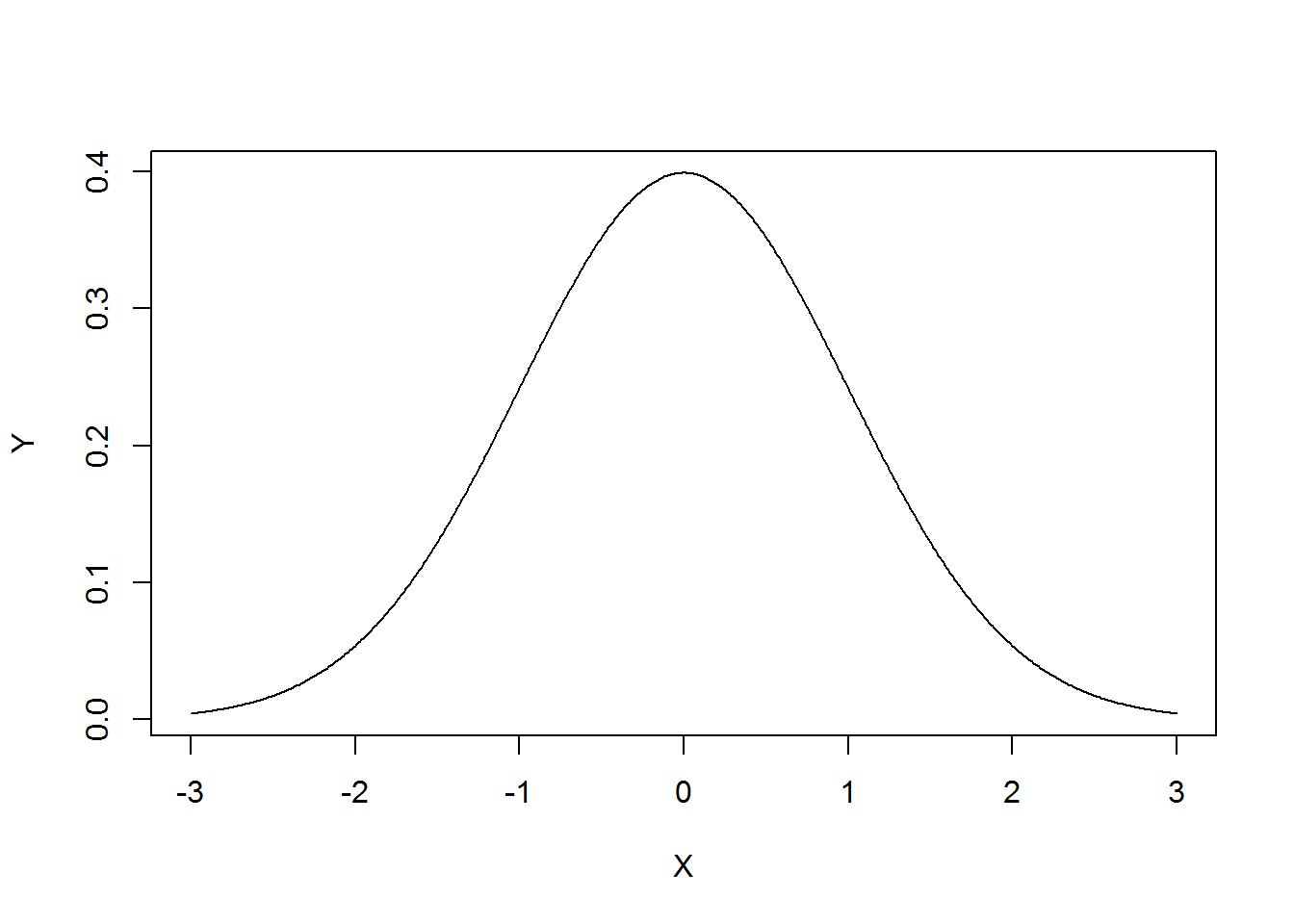# We can do the same with a chi-square distribution with 3 degrees of freedom.
X <- seq(0,10,0.01)
Y <- dchisq(X,df = 3)
plot(X,Y,type="l")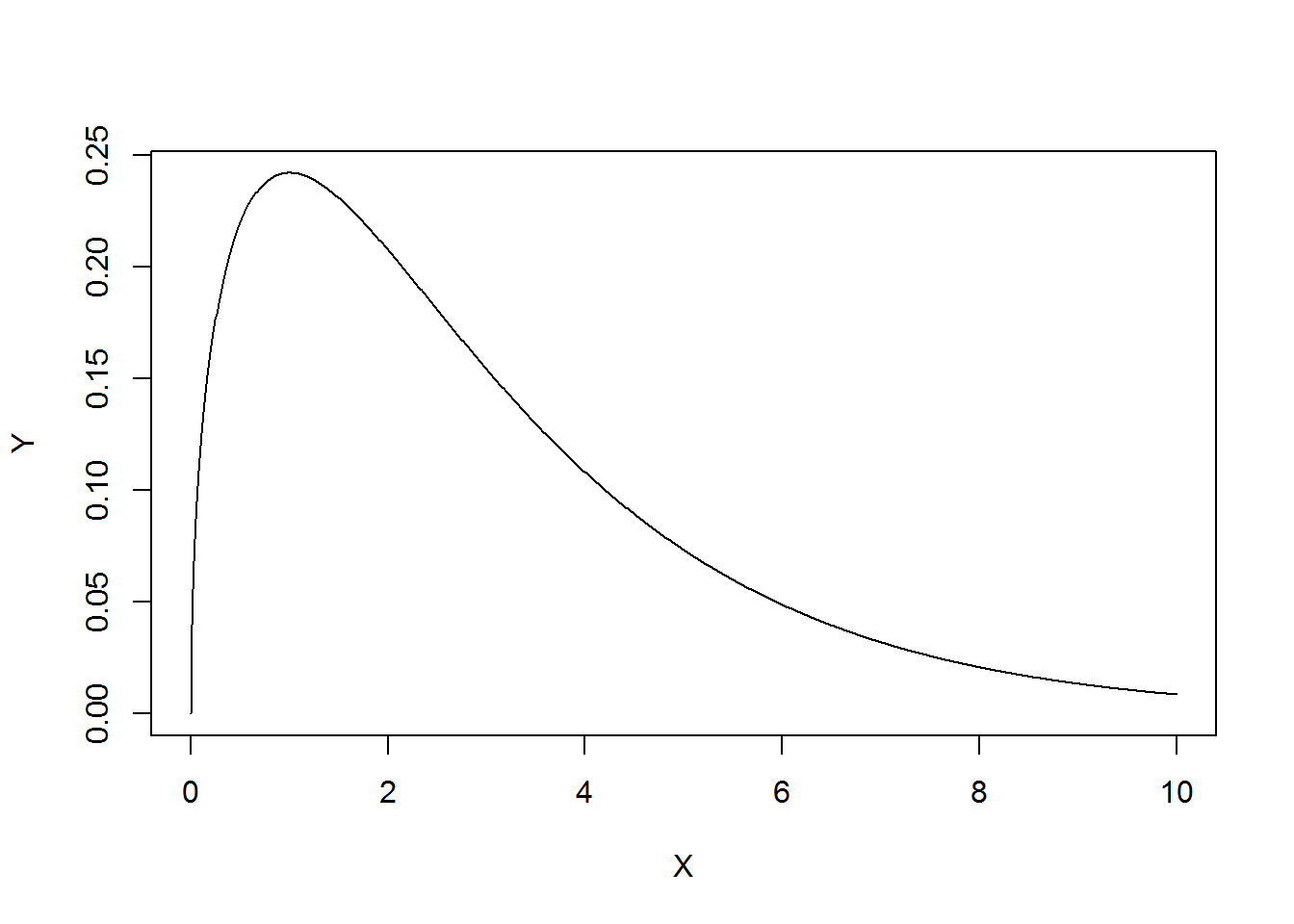# For discrete distributions such as the Binomial, you usually draw histograms
# instead of curves since we're dealing with discrete values. Here we graph the
# probability mass function for a binomial dist'n with n=10 and p=0.35.
X <- seq(0,10)
Y <- dbinom(X,size = 10,prob = 0.35)
plot(X,Y,type="h")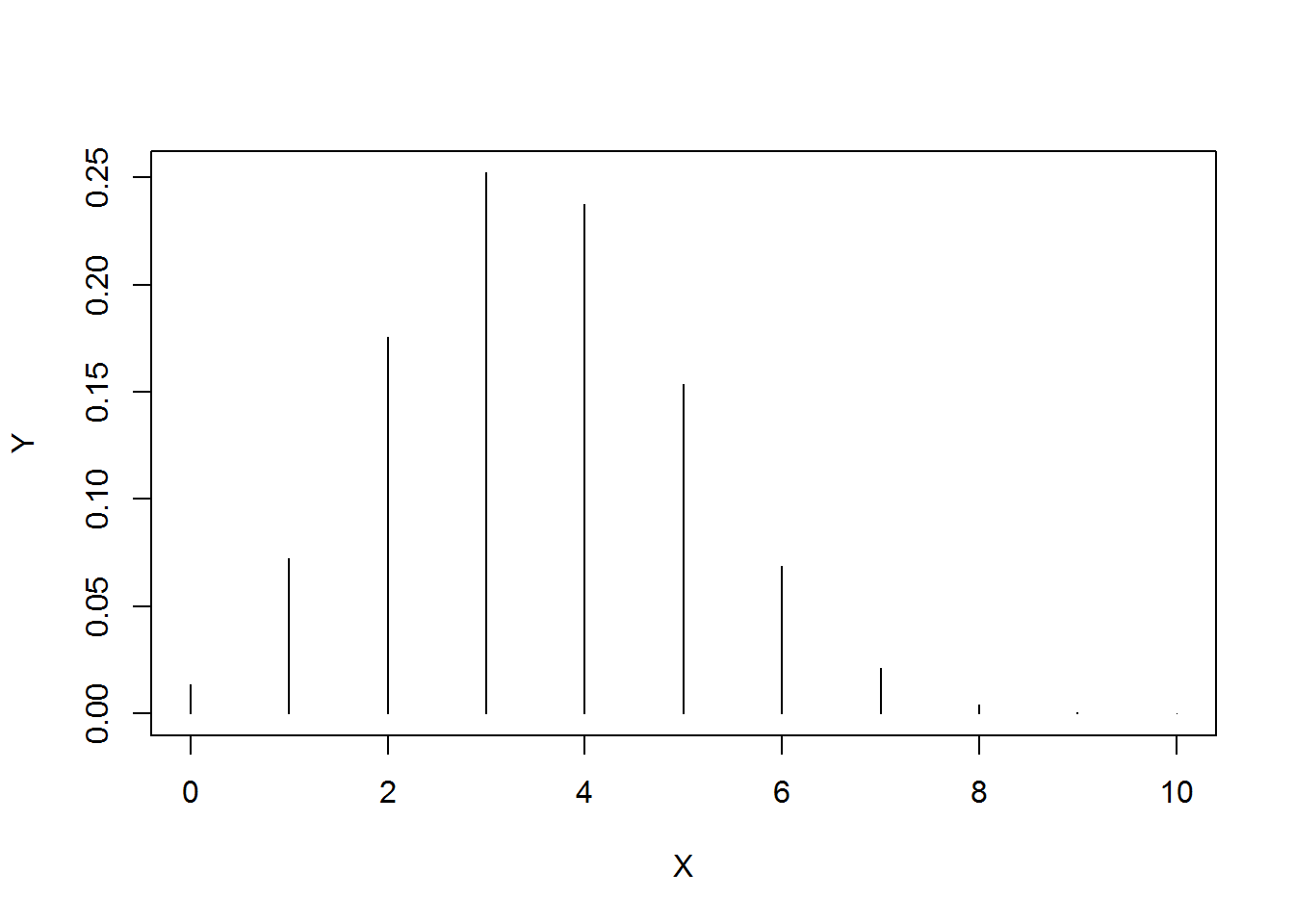# pxxx - cumulative distribution function
# This is basically the probability of a value being less than (or equal to) a
# certain point in the theoretical distribution.

# Say we have a N(100,5);
# Probability of drawing a value less than 95:
pnorm(q = 95, mean = 100, sd = 5)
##  0.1586553
# Probability of drawing a value greater than 95:
pnorm(q = 95, mean = 100, sd = 5, lower.tail = FALSE)
##  0.8413447
# or
1 - pnorm(q = 95, mean = 100, sd = 5)
##  0.8413447
# Same idea with a discrete distribution, except we say less than or equal to.
# Binomial distribution with 10 trials and probability of 0.35: b(10,0.35)
# Probability of seeing 4 or fewer "successes" out of 10 trials:
pbinom(q = 4, size = 10, prob = 0.35)
##  0.7514955
# more than 4 "successes"
pbinom(q = 4, size = 10, prob = 0.35, lower.tail = F)
##  0.2485045
# qxxx - the quantile function
# This is basically the opposite of pxxx.
# This returns the point (or the quantile) for a given probability.

# Say we have a N(100,5);
# In what "lower" quantile can we expect to see values 15% of the time:
qnorm(p = 0.15, mean = 100, sd = 5)
##  94.81783
# In what "upper" quantile can we expect to see values 15% of the time:
qnorm(p = 0.15, mean = 100, sd = 5, lower.tail = FALSE)
##  105.1822
# Say we have a b(10,0.35);
# How many successes can we expect to see 70% of the time:
qbinom(p = 0.7, size = 10, prob = 0.35)
##  4
# 4 or fewer
qbinom(p = 0.7, size = 10, prob = 0.35, lower.tail = FALSE)
##  3
# more than 3

# qnorm can be helpful when shading in areas under curves
# Normal curve for N(100,5)
X <- seq(85,115,0.01)
Y <- dnorm(X, mean = 100, sd = 5)
plot(X,Y,type="l")
# quantile for p=0.15
q <- qnorm(p = 0.15, mean = 100, sd = 5)
# create vectors of x,y coordinates for polygon function;
# rev() reverses vectors: rev(1:3) = 3 2 1
xx <- c(seq(85,q,length.out = 100),rev(seq(85,q,length = 100)))
yy <- c(rep(0,100),dnorm(rev(seq(85,q,length = 100)), mean = 100, sd = 5))
# use polygon to fill area under curve
polygon(x=xx,y=yy,col="grey")
# annotate graph
text(x = 93, y = 0.005,labels = pnorm(q,mean = 100,sd = 5))
text(x = q, y = 0.06, labels = round(q,2))